# 前言

• HashMapJavaAndroid 开发中非常常见
• 今天，我将带来HashMap 的全部源码分析，希望你们会喜欢。
1. 本文基于版本 JDK 1.7，即 Java 7
2. 关于版本 JDK 1.8，即 Java 8，具体请看文章Java源码分析：关于 HashMap 1.8 的重大更新

# 目录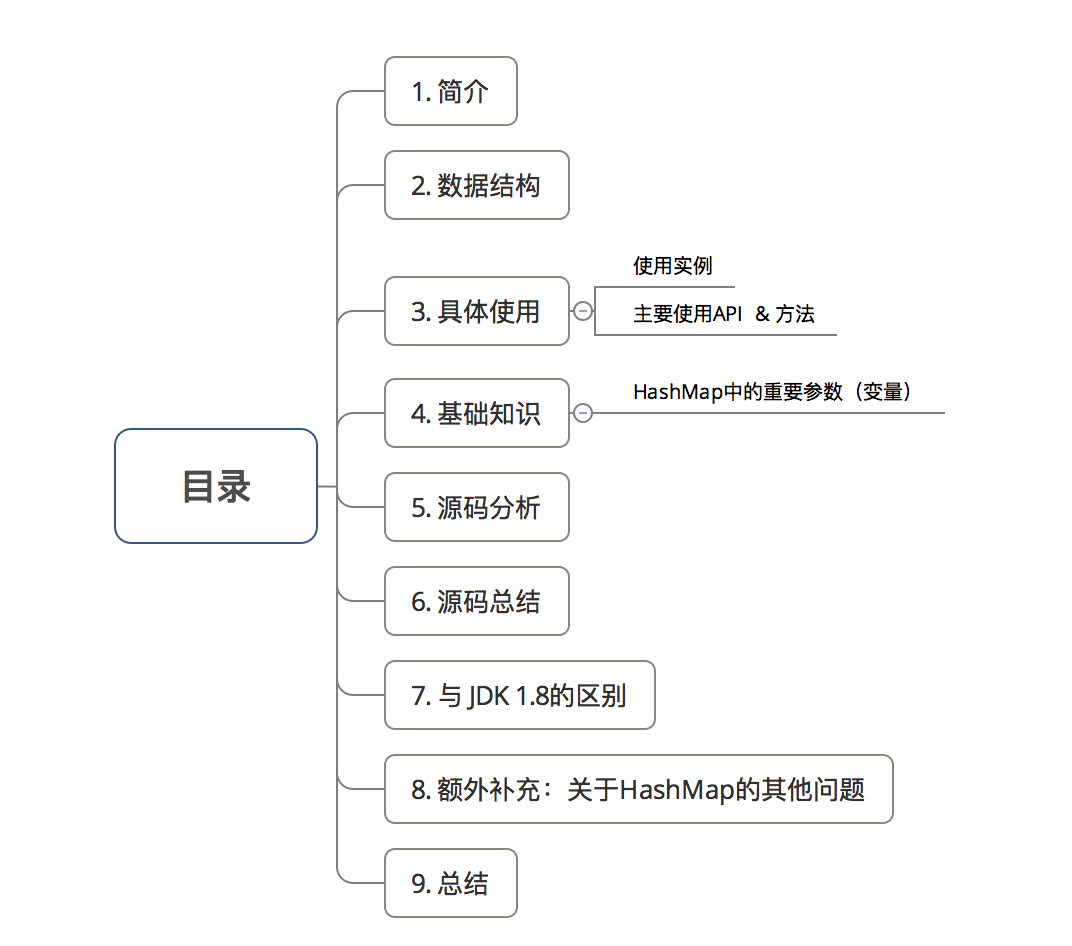# 1. 简介

• 类定义
public class HashMap<K,V>
extends AbstractMap<K,V>
implements Map<K,V>, Cloneable, Serializable

• 主要介绍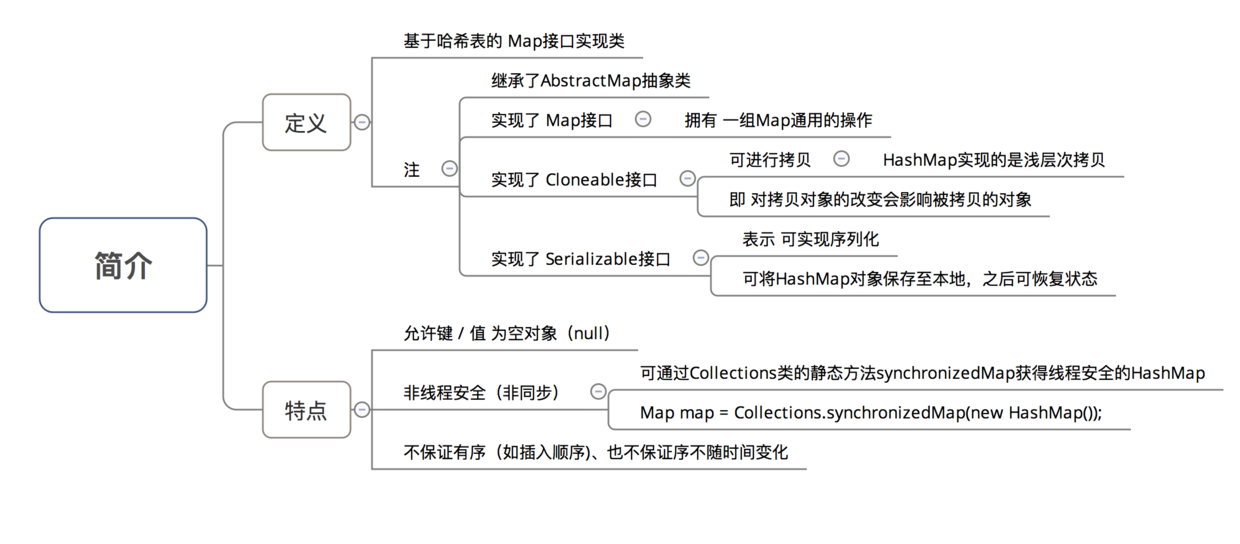• HashMap 的实现在 JDK 1.7JDK 1.8 差别较大
• 今天，我将主要讲解 JDK 1.7HashMap 的源码解析

# 2. 数据结构

### 2.1 具体描述

HashMap 采用的数据结构 = 数组（主） + 单链表（副），具体描述如下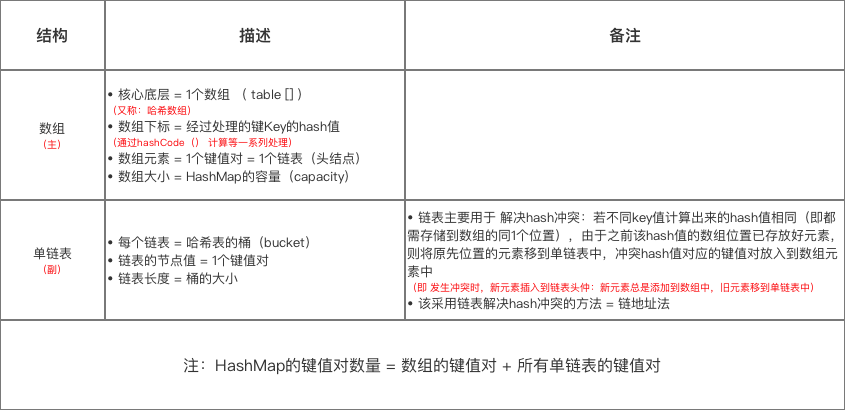### 2.2 示意图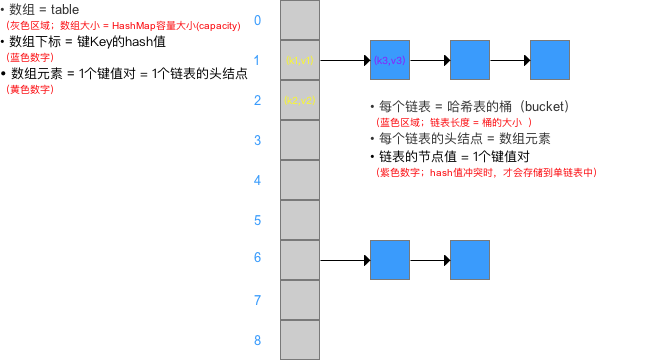### 2.3 存储流程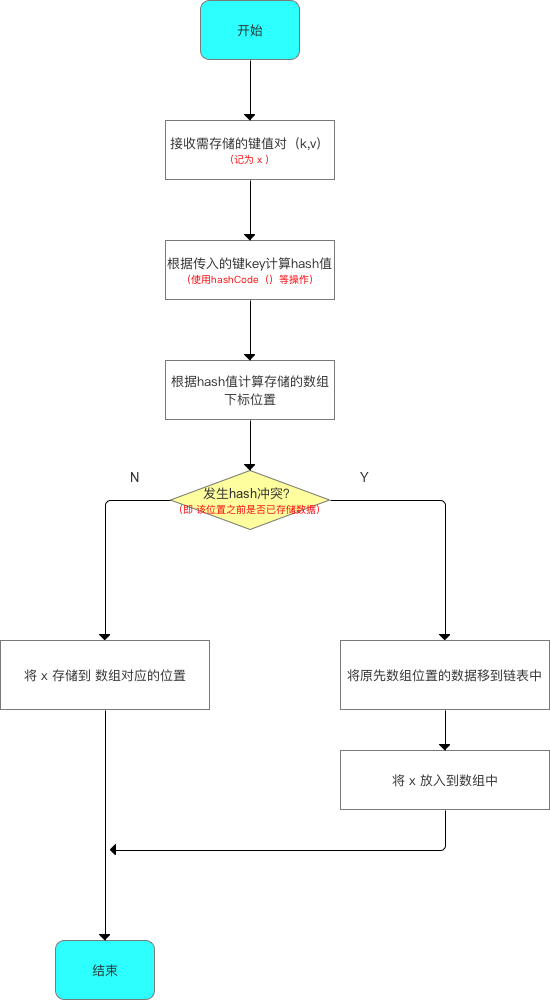### 2.4 数组元素 & 链表节点的 实现类

• HashMap中的数组元素 & 链表节点 采用 Entry类 实现，如下图所示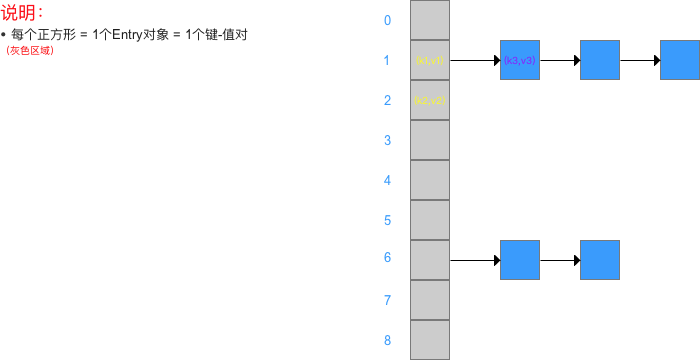1. HashMap的本质 = 1个存储Entry类对象的数组 + 多个单链表
2. Entry对象本质 = 1个映射（键 - 值对），属性包括：键（key）、值（value） & 下1节点( next) = 单链表的指针 = 也是一个Entry对象，用于解决hash冲突
• 该类的源码分析如下

/**
* Entry类实现了Map.Entry接口
* 即 实现了getKey()、getValue()、equals(Object o)和hashCode()等方法
**/
static class Entry<K,V> implements Map.Entry<K,V> {
final K key;  // 键
V value;  // 值
Entry<K,V> next; // 指向下一个节点 ，也是一个Entry对象，从而形成解决hash冲突的单链表
int hash;  // hash值

/**
* 构造方法，创建一个Entry
* 参数：哈希值h，键值k，值v、下一个节点n
*/
Entry(int h, K k, V v, Entry<K,V> n) {
value = v;
next = n;
key = k;
hash = h;
}

// 返回 与 此项 对应的键
public final K getKey() {
return key;
}

// 返回 与 此项 对应的值
public final V getValue() {
return value;
}

public final V setValue(V newValue) {
V oldValue = value;
value = newValue;
return oldValue;
}

/**
* equals（）
* 作用：判断2个Entry是否相等，必须key和value都相等，才返回true
*/
public final boolean equals(Object o) {
if (!(o instanceof Map.Entry))
return false;
Map.Entry e = (Map.Entry)o;
Object k1 = getKey();
Object k2 = e.getKey();
if (k1 == k2 || (k1 != null && k1.equals(k2))) {
Object v1 = getValue();
Object v2 = e.getValue();
if (v1 == v2 || (v1 != null && v1.equals(v2)))
return true;
}
return false;
}

/**
* hashCode（）
*/
public final int hashCode() {
return Objects.hashCode(getKey()) ^ Objects.hashCode(getValue());
}

public final String toString() {
return getKey() + "=" + getValue();
}

/**
* 当向HashMap中添加元素时，即调用put(k,v)时，
* 对已经在HashMap中k位置进行v的覆盖时，会调用此方法
* 此处没做任何处理
*/
void recordAccess(HashMap<K,V> m) {
}

/**
* 当从HashMap中删除了一个Entry时，会调用该函数
* 此处没做任何处理
*/
void recordRemoval(HashMap<K,V> m) {
}

}


# 3. 具体使用

### 3.1 主要使用API（方法、函数）

V get(Object key); // 获得指定键的值
V put(K key, V value);  // 添加键值对
void putAll(Map<? extends K, ? extends V> m);  // 将指定Map中的键值对 复制到 此Map中
V remove(Object key);  // 删除该键值对

boolean containsKey(Object key); // 判断是否存在该键的键值对；是 则返回true
boolean containsValue(Object value);  // 判断是否存在该值的键值对；是 则返回true

Set<K> keySet();  // 单独抽取key序列，将所有key生成一个Set
Collection<V> values();  // 单独value序列，将所有value生成一个Collection

void clear(); // 清除哈希表中的所有键值对
int size();  // 返回哈希表中所有 键值对的数量 = 数组中的键值对 + 链表中的键值对
boolean isEmpty(); // 判断HashMap是否为空；size == 0时 表示为 空



### 3.2 使用流程

• 在具体使用时，主要流程是：
1. 声明1个 HashMap的对象
2. HashMap 添加数据（成对 放入 键 - 值对）
3. 获取 HashMap 的某个数据
4. 获取 HashMap 的全部数据：遍历HashMap
• 示例代码
import java.util.Collection;
import java.util.HashMap;
import java.util.Iterator;
import java.util.Map;
import java.util.Set;

public class HashMapTest {

public static void main(String[] args) {
/**
* 1. 声明1个 HashMap的对象
*/
Map<String, Integer> map = new HashMap<String, Integer>();

/**
* 2. 向HashMap添加数据（成对 放入 键 - 值对）
*/
map.put("Android", 1);
map.put("Java", 2);
map.put("iOS", 3);
map.put("数据挖掘", 4);
map.put("产品经理", 5);

/**
* 3. 获取 HashMap 的某个数据
*/
System.out.println("key = 产品经理时的值为：" + map.get("产品经理"));

/**
* 4. 获取 HashMap 的全部数据：遍历HashMap
* 核心思想：
* 步骤1：获得key-value对（Entry） 或 key 或 value的Set集合
* 步骤2：遍历上述Set集合(使用for循环 、 迭代器（Iterator）均可)
* 方法共有3种：分别针对 key-value对（Entry） 或 key 或 value
*/

// 方法1：获得key-value的Set集合 再遍历
System.out.println("方法1");
// 1. 获得key-value对（Entry）的Set集合
Set<Map.Entry<String, Integer>> entrySet = map.entrySet();

// 2. 遍历Set集合，从而获取key-value
// 2.1 通过for循环
for(Map.Entry<String, Integer> entry : entrySet){
System.out.print(entry.getKey());
System.out.println(entry.getValue());
}
System.out.println("----------");
// 2.2 通过迭代器：先获得key-value对（Entry）的Iterator，再循环遍历
Iterator iter1 = entrySet.iterator();
while (iter1.hasNext()) {
// 遍历时，需先获取entry，再分别获取key、value
Map.Entry entry = (Map.Entry) iter1.next();
System.out.print((String) entry.getKey());
System.out.println((Integer) entry.getValue());
}

// 方法2：获得key的Set集合 再遍历
System.out.println("方法2");

// 1. 获得key的Set集合
Set<String> keySet = map.keySet();

// 2. 遍历Set集合，从而获取key，再获取value
// 2.1 通过for循环
for(String key : keySet){
System.out.print(key);
System.out.println(map.get(key));
}

System.out.println("----------");

// 2.2 通过迭代器：先获得key的Iterator，再循环遍历
Iterator iter2 = keySet.iterator();
String key = null;
while (iter2.hasNext()) {
key = (String)iter2.next();
System.out.print(key);
System.out.println(map.get(key));
}

// 方法3：获得value的Set集合 再遍历
System.out.println("方法3");

// 1. 获得value的Set集合
Collection valueSet = map.values();

// 2. 遍历Set集合，从而获取value
// 2.1 获得values 的Iterator
Iterator iter3 = valueSet.iterator();
// 2.2 通过遍历，直接获取value
while (iter3.hasNext()) {
System.out.println(iter3.next());
}

}

}

// 注：对于遍历方式，推荐使用针对 key-value对（Entry）的方式：效率高
// 原因：
// 1. 对于 遍历keySet 、valueSet，实质上 = 遍历了2次：1 = 转为 iterator 迭代器遍历、2 = 从 HashMap 中取出 key 的 value 操作（通过 key 值 hashCode 和 equals 索引）
// 2. 对于 遍历 entrySet ，实质 = 遍历了1次 = 获取存储实体Entry（存储了key 和 value ）

• 运行结果
方法1
Java2
iOS3

Android1

----------
Java2
iOS3

Android1

Java2
iOS3

Android1

----------
Java2
iOS3

Android1

2
3
4
1
5


# 4. 基础知识：HashMap中的重要参数（变量）

• 在进行真正的源码分析前，先讲解HashMap中的重要参数（变量）
• HashMap中的主要参数 = 容量、加载因子、扩容阈值
• 具体介绍如下
// 1. 容量（capacity）： HashMap中数组的长度
// a. 容量范围：必须是2的幂 & <最大容量（2的30次方）
// b. 初始容量 = 哈希表创建时的容量
// 默认容量 = 16 = 1<<4 = 00001中的1向左移4位 = 10000 = 十进制的2^4=16
static final int DEFAULT_INITIAL_CAPACITY = 1 << 4;
// 最大容量 =  2的30次方（若传入的容量过大，将被最大值替换）
static final int MAXIMUM_CAPACITY = 1 << 30;

// a. 加载因子越大、填满的元素越多 = 空间利用率高、但冲突的机会加大、查找效率变低（因为链表变长了）
// b. 加载因子越小、填满的元素越少 = 空间利用率小、冲突的机会减小、查找效率高（链表不长）
// 实际加载因子
// 默认加载因子 = 0.75
static final float DEFAULT_LOAD_FACTOR = 0.75f;

// 3. 扩容阈值（threshold）：当哈希表的大小 ≥ 扩容阈值时，就会扩容哈希表（即扩充HashMap的容量）
// a. 扩容 = 对哈希表进行resize操作（即重建内部数据结构），从而哈希表将具有大约两倍的桶数
// b. 扩容阈值 = 容量 x 加载因子
int threshold;

// 4. 其他
// 存储数据的Entry类型 数组，长度 = 2的幂
// HashMap的实现方式 = 拉链法，Entry数组上的每个元素本质上是一个单向链表
transient Entry<K,V>[] table = (Entry<K,V>[]) EMPTY_TABLE;
// HashMap的大小，即 HashMap中存储的键值对的数量
transient int size;


• 参数示意图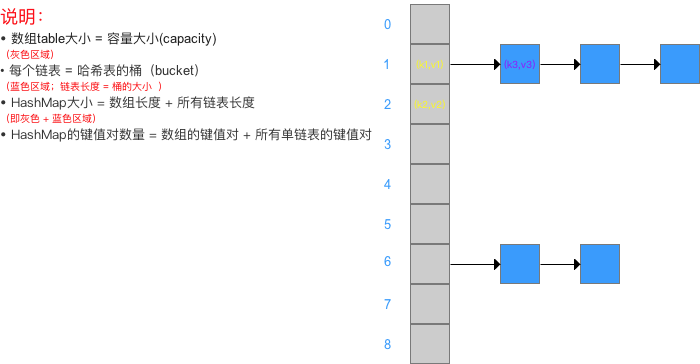• 此处 详细说明 加载因子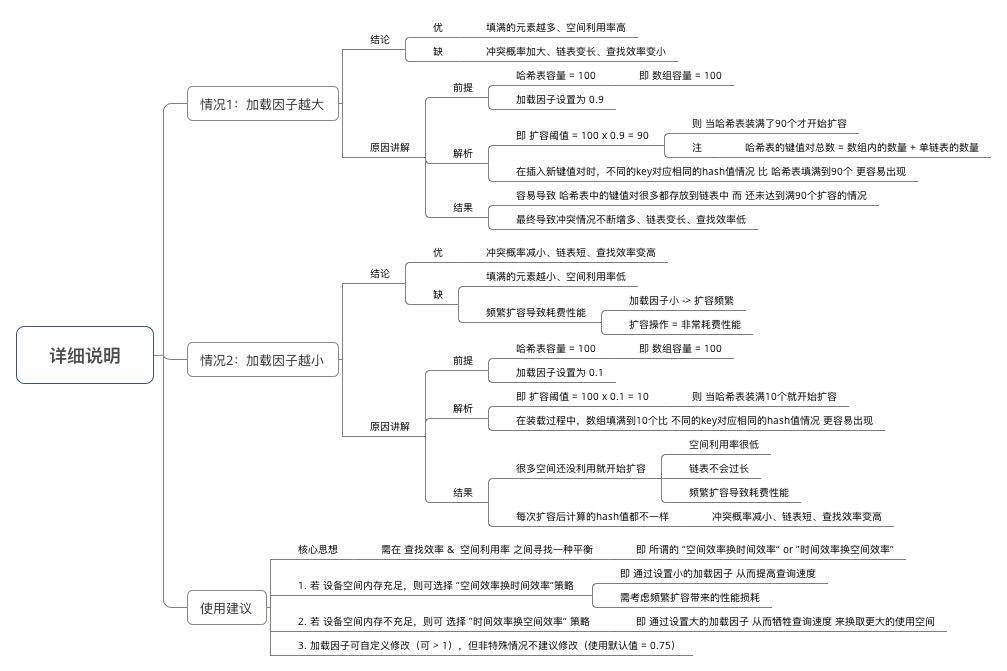# 5. 源码分析

• 本次的源码分析主要是根据 使用步骤 进行相关函数的详细分析
• 主要分析内容如下：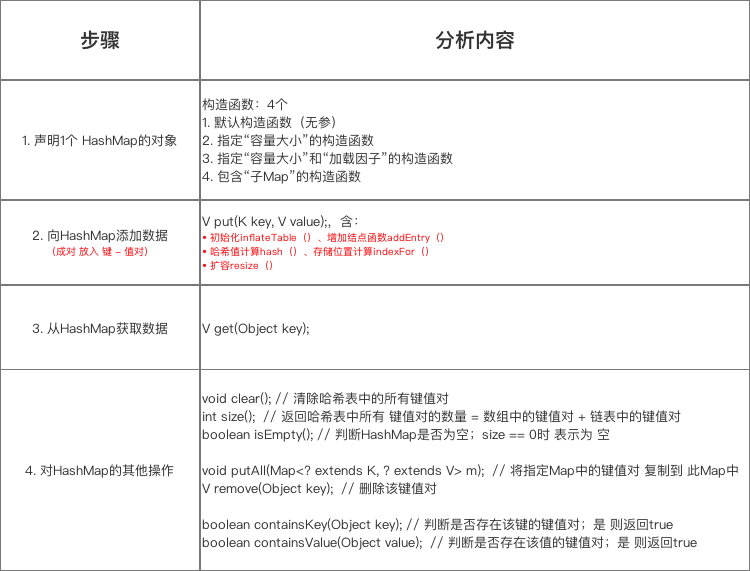• 下面，我将对每个步骤内容的主要方法进行详细分析

### 步骤1：声明1个 HashMap的对象

/**
* 函数使用原型
*/
Map<String,Integer> map = new HashMap<String,Integer>();

/**
* 源码分析：主要是HashMap的构造函数 = 4个
* 仅贴出关于HashMap构造函数的源码
*/
public class HashMap<K,V>
extends AbstractMap<K,V>
implements Map<K,V>, Cloneable, Serializable{

// 省略上节阐述的参数

/**
* 构造函数1：默认构造函数（无参）
* 加载因子 & 容量 = 默认 = 0.75、16
*/
public HashMap() {
// 实际上是调用构造函数3：指定“容量大小”和“加载因子”的构造函数
// 传入的指定容量 & 加载因子 = 默认
}

/**
* 构造函数2：指定“容量大小”的构造函数
* 加载因子 = 默认 = 0.75 、容量 = 指定大小
*/
public HashMap(int initialCapacity) {
// 实际上是调用指定“容量大小”和“加载因子”的构造函数
// 只是在传入的加载因子参数 = 默认加载因子

}

/**
* 构造函数3：指定“容量大小”和“加载因子”的构造函数
* 加载因子 & 容量 = 自己指定
*/
public HashMap(int initialCapacity, float loadFactor) {

// HashMap的最大容量只能是MAXIMUM_CAPACITY，哪怕传入的 > 最大容量
if (initialCapacity > MAXIMUM_CAPACITY)
initialCapacity = MAXIMUM_CAPACITY;

// 设置 加载因子
// 设置 扩容阈值 = 初始容量
// 注：此处不是真正的阈值，是为了扩展table，该阈值后面会重新计算，下面会详细讲解
threshold = initialCapacity;

init(); // 一个空方法用于未来的子对象扩展
}

/**
* 构造函数4：包含“子Map”的构造函数
* 即 构造出来的HashMap包含传入Map的映射关系
* 加载因子 & 容量 = 默认
*/

public HashMap(Map<? extends K, ? extends V> m) {

// 设置容量大小 & 加载因子 = 默认
this(Math.max((int) (m.size() / DEFAULT_LOAD_FACTOR) + 1,

// 该方法用于初始化 数组 & 阈值，下面会详细说明
inflateTable(threshold);

// 将传入的子Map中的全部元素逐个添加到HashMap中
putAllForCreate(m);
}
}

• 注：
1. 此处仅用于接收初始容量大小（capacity）、加载因子(Load factor)，但仍无真正初始化哈希表，即初始化存储数组table
2. 此处先给出结论：真正初始化哈希表（初始化存储数组table）是在第1次添加键值对时，即第1次调用put（）时。下面会详细说明

### 步骤2：向HashMap添加数据（成对 放入 键 - 值对）

• 添加数据的流程如下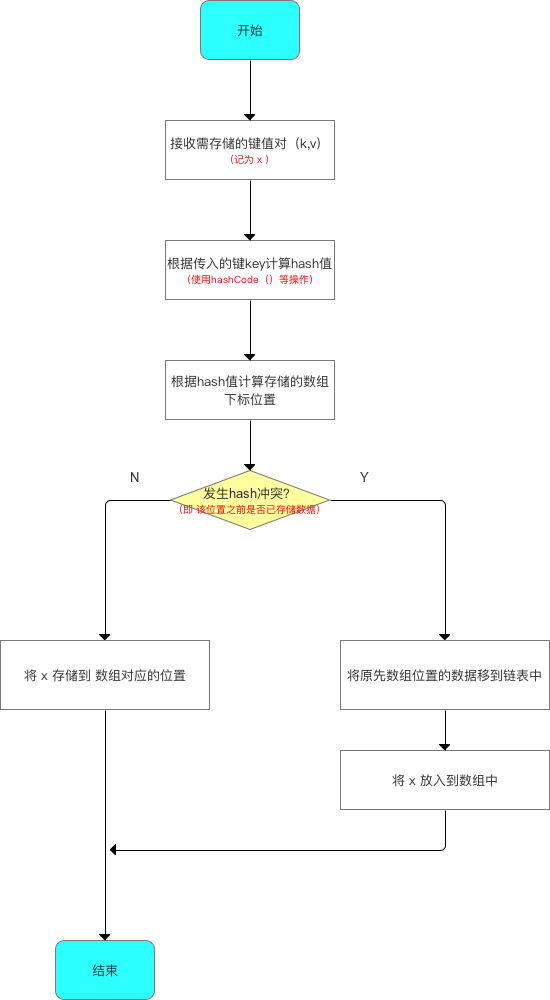• 源码分析
 /**
* 函数使用原型
*/
map.put("Android", 1);
map.put("Java", 2);
map.put("iOS", 3);
map.put("数据挖掘", 4);
map.put("产品经理", 5);

/**
* 源码分析：主要分析： HashMap的put函数
*/
public V put(K key, V value)
（分析1）// 1. 若 哈希表未初始化（即 table为空)
// 则使用 构造函数时设置的阈值(即初始容量) 初始化 数组table
if (table == EMPTY_TABLE) {
inflateTable(threshold);
}
// 2. 判断key是否为空值null
（分析2）// 2.1 若key == null，则将该键-值 存放到数组table 中的第1个位置，即table 
// （本质：key = Null时，hash值 = 0，故存放到table中）
// 该位置永远只有1个value，新传进来的value会覆盖旧的value
if (key == null)
return putForNullKey(value);

（分析3） // 2.2 若 key ≠ null，则计算存放数组 table 中的位置（下标、索引）
// a. 根据键值key计算hash值
int hash = hash(key);
// b. 根据hash值 最终获得 key对应存放的数组Table中位置
int i = indexFor(hash, table.length);

// 3. 判断该key对应的值是否已存在（通过遍历 以该数组元素为头结点的链表 逐个判断）
for (Entry<K,V> e = table[i]; e != null; e = e.next) {
Object k;
（分析4）// 3.1 若该key已存在（即 key-value已存在 ），则用 新value 替换 旧value
if (e.hash == hash && ((k = e.key) == key || key.equals(k))) {
V oldValue = e.value;
e.value = value;
e.recordAccess(this);
return oldValue; //并返回旧的value
}
}

modCount++;

（分析5）// 3.2 若 该key不存在，则将“key-value”添加到table中
return null;
}

• 根据源码分析所作出的流程图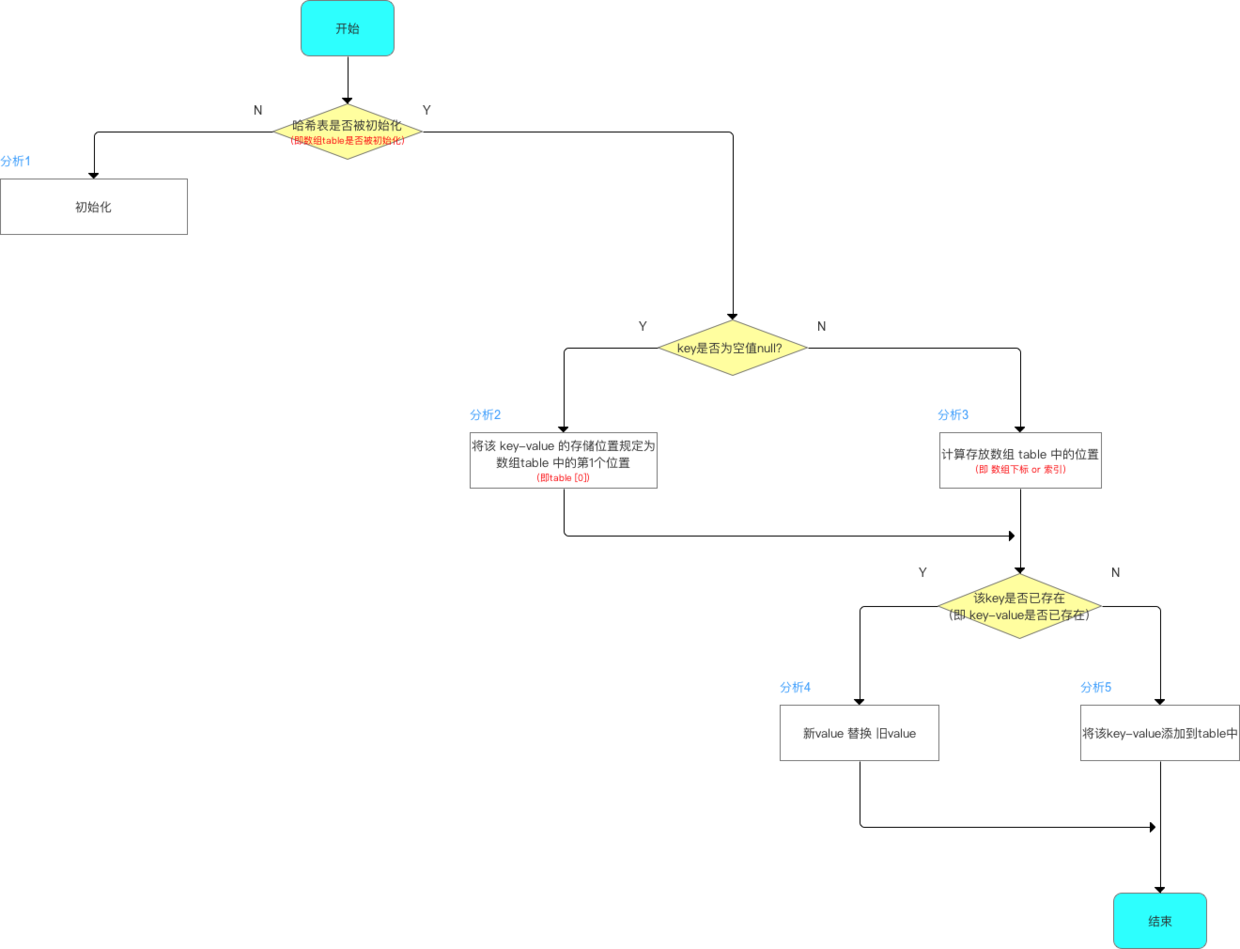• 下面，我将根据上述流程的5个分析点进行详细讲解

### 分析1：初始化哈希表

   /**
* 函数使用原型
*/
if (table == EMPTY_TABLE) {
inflateTable(threshold);
}

/**
* 源码分析：inflateTable(threshold);
*/
private void inflateTable(int toSize) {

// 1. 将传入的容量大小转化为：>传入容量大小的最小的2的次幂
// 即如果传入的是容量大小是19，那么转化后，初始化容量大小为32（即2的5次幂）
int capacity = roundUpToPowerOf2(toSize);->>分析1

// 2. 重新计算阈值 threshold = 容量 * 加载因子
threshold = (int) Math.min(capacity * loadFactor, MAXIMUM_CAPACITY + 1);

// 3. 使用计算后的初始容量（已经是2的次幂） 初始化数组table（作为数组长度）
// 即 哈希表的容量大小 = 数组大小（长度）
table = new Entry[capacity]; //用该容量初始化table

initHashSeedAsNeeded(capacity);
}

/**
* 分析1：roundUpToPowerOf2(toSize)
* 作用：将传入的容量大小转化为：>传入容量大小的最小的2的幂
* 特别注意：容量大小必须为2的幂，该原因在下面的讲解会详细分析
*/

private static int roundUpToPowerOf2(int number) {

//若 容量超过了最大值，初始化容量设置为最大值 ；否则，设置为：>传入容量大小的最小的2的次幂
return number >= MAXIMUM_CAPACITY  ?
MAXIMUM_CAPACITY  : (number > 1) ? Integer.highestOneBit((number - 1) << 1) : 1;

• 再次强调：真正初始化哈希表（初始化存储数组table）是在第1次添加键值对时，即第1次调用put（）

### 分析2：当 key ==null时，将该 key-value 的存储位置规定为数组table 中的第1个位置，即table 

   /**
* 函数使用原型
*/
if (key == null)
return putForNullKey(value);

/**
* 源码分析：putForNullKey(value)
*/
private V putForNullKey(V value) {
// 遍历以table为首的链表，寻找是否存在key==null 对应的键值对
// 1. 若有：则用新value 替换 旧value；同时返回旧的value值
for (Entry<K,V> e = table; e != null; e = e.next) {
if (e.key == null) {
V oldValue = e.value;
e.value = value;
e.recordAccess(this);
return oldValue;
}
}
modCount++;

// 注：
// a. addEntry（）的第1个参数 = hash值 = 传入0
// b. 即 说明：当key = null时，也有hash值 = 0，所以HashMap的key 可为null
// c. 对比HashTable，由于HashTable对key直接hashCode（），若key为null时，会抛出异常，所以HashTable的key不可为null
return null;

}


• HashMap的键key 可为null（区别于 HashTablekey 不可为null
• HashMap的键key 可为null且只能为1个，但值value可为null且为多个

### 分析3：计算存放数组 table 中的位置（即 数组下标 or 索引）

   /**
* 函数使用原型
* 主要分为2步：计算hash值、根据hash值再计算得出最后数组位置
*/
// a. 根据键值key计算hash值 ->> 分析1
int hash = hash(key);
// b. 根据hash值 最终获得 key对应存放的数组Table中位置 ->> 分析2
int i = indexFor(hash, table.length);

/**
* 源码分析1：hash(key)
* 该函数在JDK 1.7 和 1.8 中的实现不同，但原理一样 = 扰动函数 = 使得根据key生成的哈希码（hash值）分布更加均匀、更具备随机性，避免出现hash值冲突（即指不同key但生成同1个hash值）
* JDK 1.7 做了9次扰动处理 = 4次位运算 + 5次异或运算
* JDK 1.8 简化了扰动函数 = 只做了2次扰动 = 1次位运算 + 1次异或运算
*/

// JDK 1.7实现：将 键key 转换成 哈希码（hash值）操作  = 使用hashCode() + 4次位运算 + 5次异或运算（9次扰动）
static final int hash(int h) {
h ^= k.hashCode();
h ^= (h >>> 20) ^ (h >>> 12);
return h ^ (h >>> 7) ^ (h >>> 4);
}

// JDK 1.8实现：将 键key 转换成 哈希码（hash值）操作 = 使用hashCode() + 1次位运算 + 1次异或运算（2次扰动）
// 1. 取hashCode值： h = key.hashCode()
//  2. 高位参与低位的运算：h ^ (h >>> 16)
static final int hash(Object key) {
int h;
return (key == null) ? 0 : (h = key.hashCode()) ^ (h >>> 16);
// a. 当key = null时，hash值 = 0，所以HashMap的key 可为null
// 注：对比HashTable，HashTable对key直接hashCode（），若key为null时，会抛出异常，所以HashTable的key不可为null
// b. 当key ≠ null时，则通过先计算出 key的 hashCode()（记为h），然后 对哈希码进行 扰动处理： 按位 异或（^） 哈希码自身右移16位后的二进制
}

/**
* 函数源码分析2：indexFor(hash, table.length)
* JDK 1.8中实际上无该函数，但原理相同，即具备类似作用的函数
*/
static int indexFor(int h, int length) {
return h & (length-1);
// 将对哈希码扰动处理后的结果 与运算(&) （数组长度-1），最终得到存储在数组table的位置（即数组下标、索引）
}

• 总结 计算存放在数组 table 中的位置（即数组下标、索引）的过程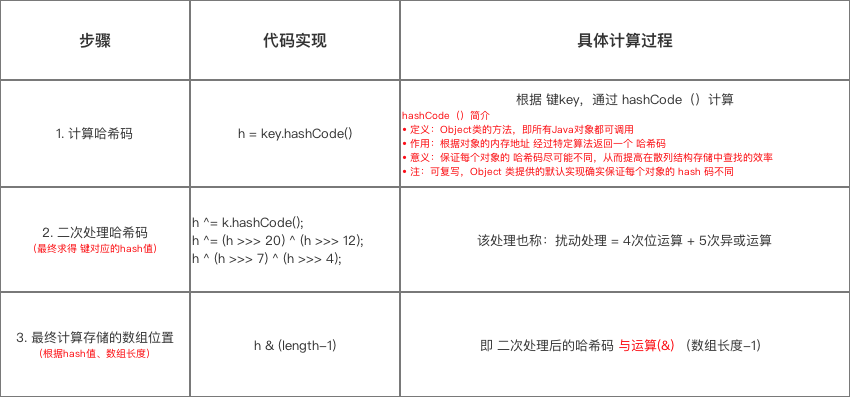1. 为什么不直接采用经过hashCode（）处理的哈希码 作为 存储数组table的下标位置？
2. 为什么采用 哈希码 与运算(&) （数组长度-1） 计算数组下标？
3. 为什么在计算数组下标前，需对哈希码进行二次处理：扰动处理？

### 问题1：为什么不直接采用经过hashCode（）处理的哈希码 作为 存储数组table的下标位置？

• 结论：容易出现 哈希码 与 数组大小范围不匹配的情况，即 计算出来的哈希码可能 不在数组大小范围内，从而导致无法匹配存储位置
• 原因描述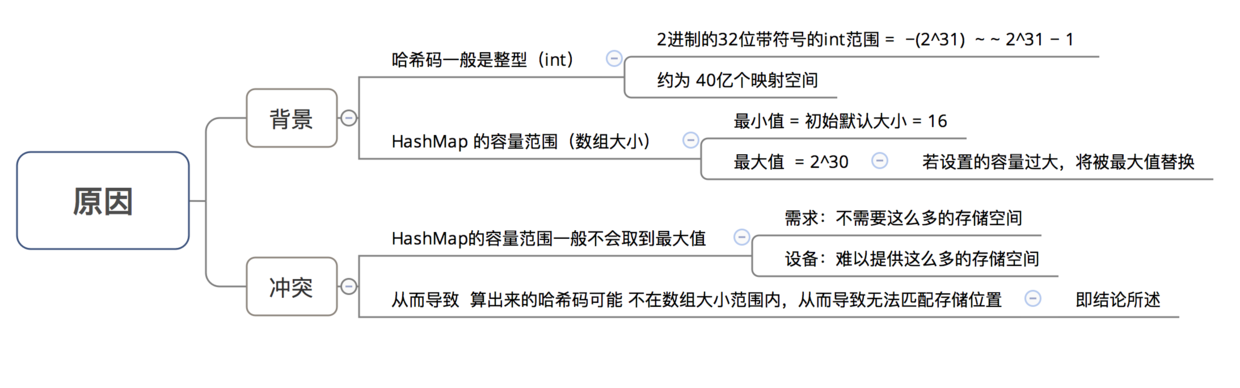• 为了解决 “哈希码与数组大小范围不匹配” 的问题，HashMap给出了解决方案：哈希码 与运算（&） （数组长度-1）；请继续问题2

### 问题2：为什么采用 哈希码 与运算(&) （数组长度-1） 计算数组下标？

• 结论：根据HashMap的容量大小（数组长度），按需取 哈希码一定数量的低位 作为存储的数组下标位置，从而 解决 “哈希码与数组大小范围不匹配” 的问题

• 具体解决方案描述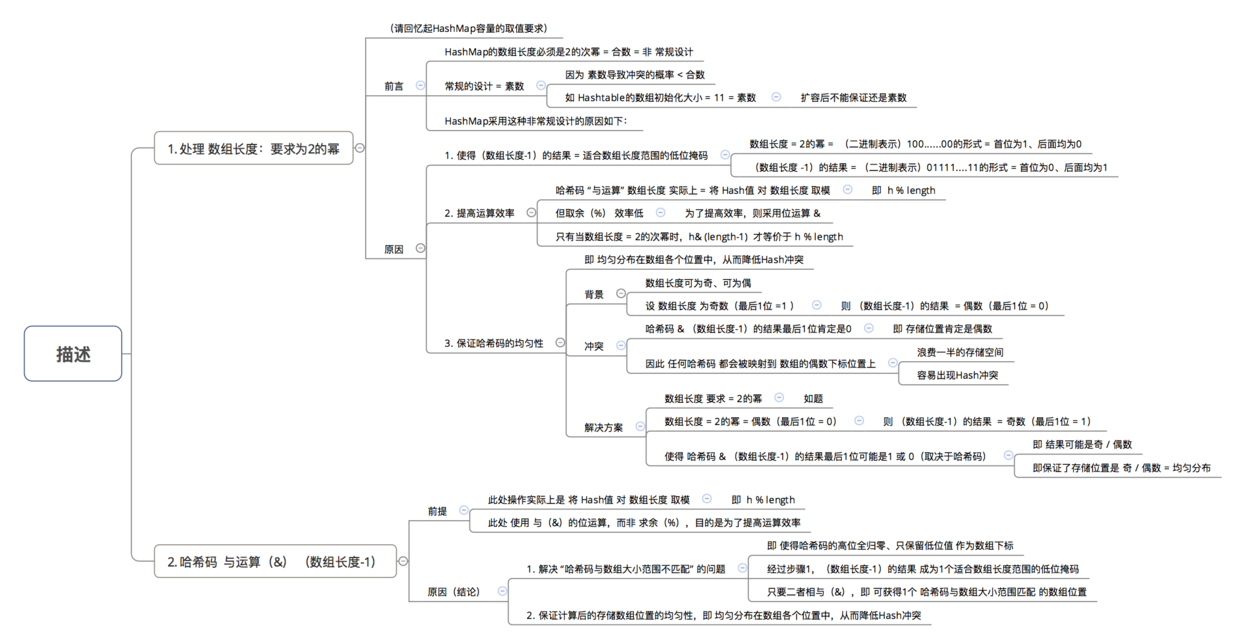### 问题3：为什么在计算数组下标前，需对哈希码进行二次处理：扰动处理？

• 结论：加大哈希码低位的随机性，使得分布更均匀，从而提高对应数组存储下标位置的随机性 & 均匀性，最终减少Hash冲突

• 具体描述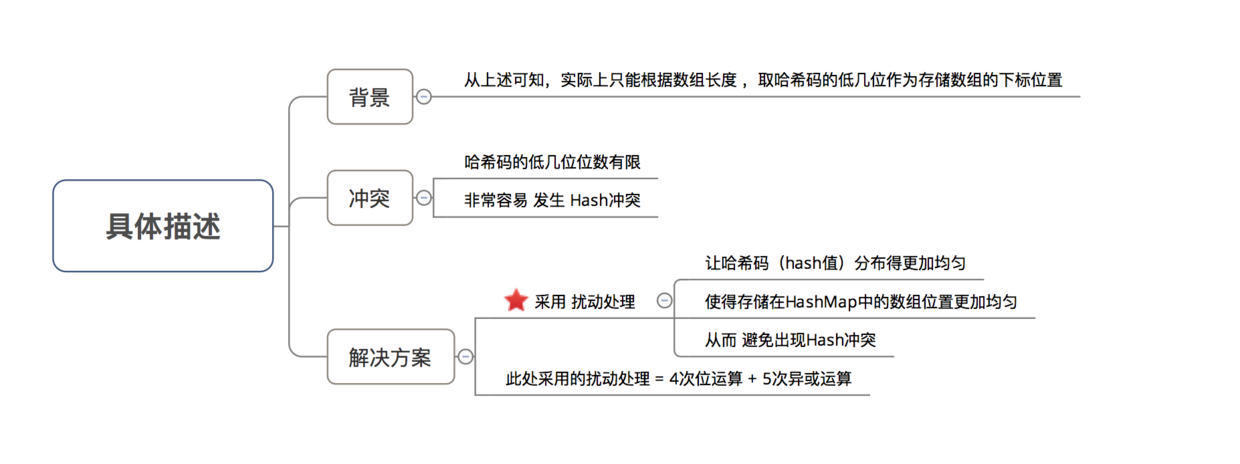### 分析4：若对应的key已存在，则 使用 新value 替换 旧value

   /**
* 函数使用原型
*/
// 2. 判断该key对应的值是否已存在（通过遍历 以该数组元素为头结点的链表 逐个判断）
for (Entry<K,V> e = table[i]; e != null; e = e.next) {
Object k;
// 2.1 若该key已存在（即 key-value已存在 ），则用 新value 替换 旧value
if (e.hash == hash && ((k = e.key) == key || key.equals(k))) {
V oldValue = e.value;
e.value = value;
e.recordAccess(this);
return oldValue; //并返回旧的value
}
}

modCount++;

// 2.2 若 该key不存在，则将“key-value”添加到table中
return null;

• 此处无复杂的源码分析，但此处的分析点主要有2个：替换流程 & key是否存在（即key值的对比）

#### 分析1：替换流程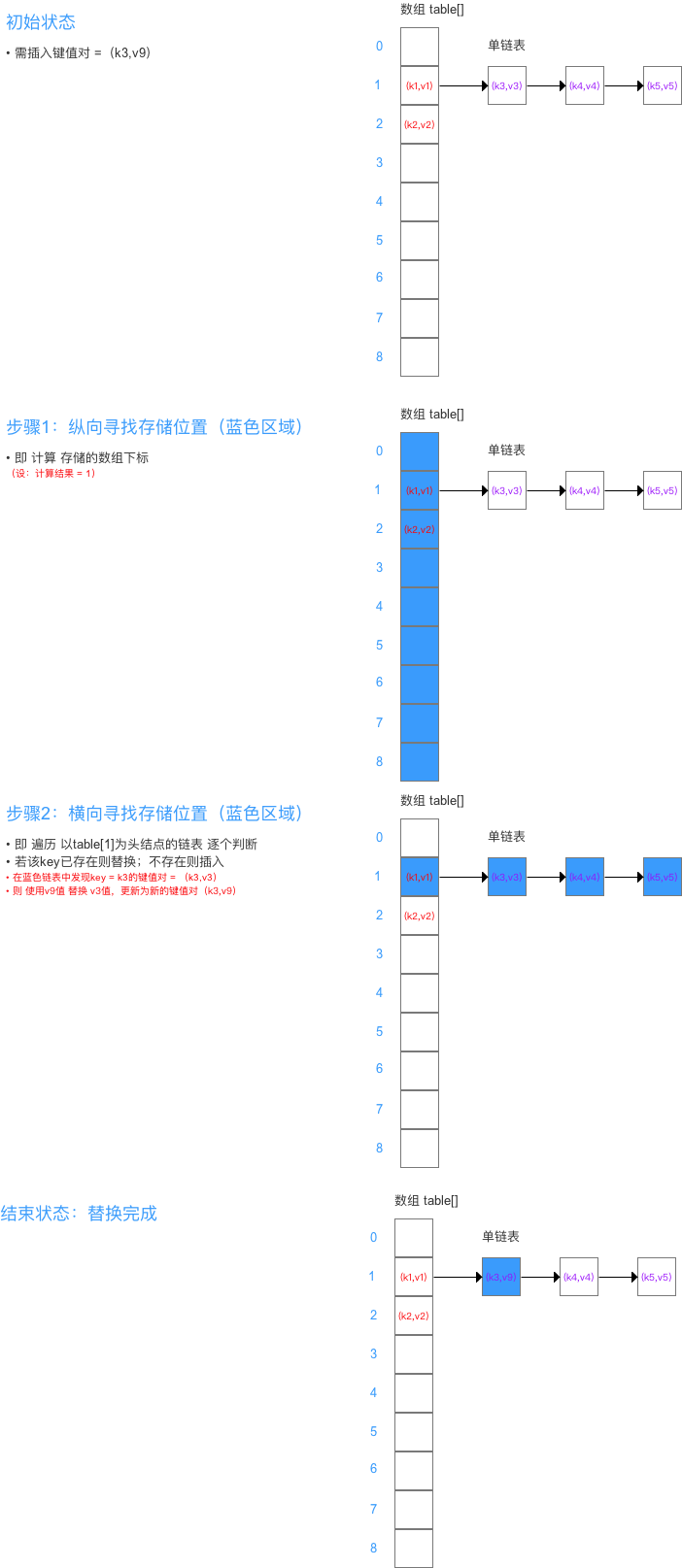#### 分析2：key值的比较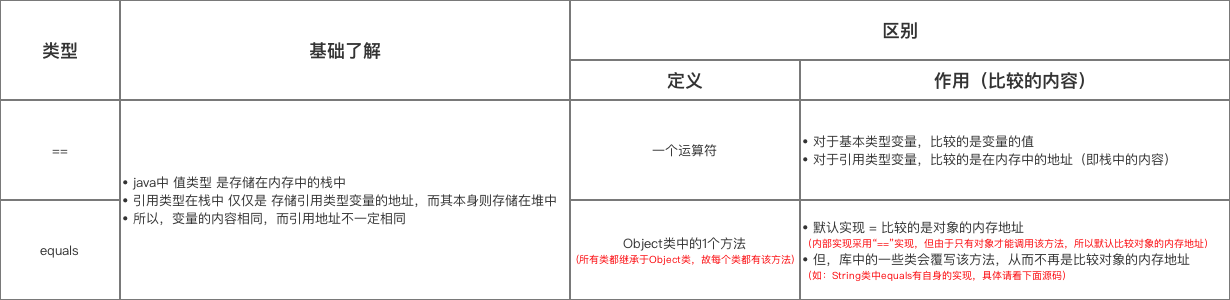### 分析5：若对应的key不存在，则将该“key-value”添加到数组table的对应位置中

• 函数源码分析如下
      /**
* 函数使用原型
*/
// 2. 判断该key对应的值是否已存在
for (Entry<K,V> e = table[i]; e != null; e = e.next) {
Object k;
// 2.1 若该key对应的值已存在，则用新的value取代旧的value
if (e.hash == hash && ((k = e.key) == key || key.equals(k))) {
V oldValue = e.value;
e.value = value;
e.recordAccess(this);
return oldValue;
}
}

modCount++;

// 2.2 若 该key对应的值不存在，则将“key-value”添加到table中

/**
* 作用：添加键值对（Entry ）到 HashMap中
*/
void addEntry(int hash, K key, V value, int bucketIndex) {
// 参数3 = 插入数组table的索引位置 = 数组下标

// 1. 插入前，先判断容量是否足够
// 1.1 若不足够，则进行扩容（2倍）、重新计算Hash值、重新计算存储数组下标
if ((size >= threshold) && (null != table[bucketIndex])) {
resize(2 * table.length); // a. 扩容2倍  --> 分析1
hash = (null != key) ? hash(key) : 0;  // b. 重新计算该Key对应的hash值
bucketIndex = indexFor(hash, table.length);  // c. 重新计算该Key对应的hash值的存储数组下标位置
}

// 1.2 若容量足够，则创建1个新的数组元素（Entry） 并放入到数组中--> 分析2
createEntry(hash, key, value, bucketIndex);
}

/**
* 分析1：resize(2 * table.length)
* 作用：当容量不足时（容量 > 阈值），则扩容（扩到2倍）
*/
void resize(int newCapacity) {

// 1. 保存旧数组（old table）
Entry[] oldTable = table;

// 2. 保存旧容量（old capacity ），即数组长度
int oldCapacity = oldTable.length;

// 3. 若旧容量已经是系统默认最大容量了，那么将阈值设置成整型的最大值，退出
if (oldCapacity == MAXIMUM_CAPACITY) {
threshold = Integer.MAX_VALUE;
return;
}

// 4. 根据新容量（2倍容量）新建1个数组，即新table
Entry[] newTable = new Entry[newCapacity];

// 5. 将旧数组上的数据（键值对）转移到新table中，从而完成扩容 ->>分析1.1
transfer(newTable);

// 6. 新数组table引用到HashMap的table属性上
table = newTable;

// 7. 重新设置阈值
}

/**
* 分析1.1：transfer(newTable);
* 作用：将旧数组上的数据（键值对）转移到新table中，从而完成扩容
* 过程：按旧链表的正序遍历链表、在新链表的头部依次插入
*/
void transfer(Entry[] newTable) {
// 1. src引用了旧数组
Entry[] src = table;

// 2. 获取新数组的大小 = 获取新容量大小
int newCapacity = newTable.length;

// 3. 通过遍历 旧数组，将旧数组上的数据（键值对）转移到新数组中
for (int j = 0; j < src.length; j++) {
// 3.1 取得旧数组的每个元素
Entry<K,V> e = src[j];
if (e != null) {
// 3.2 释放旧数组的对象引用（for循环后，旧数组不再引用任何对象）
src[j] = null;

do {
// 3.3 遍历 以该数组元素为首 的链表
// 注：转移链表时，因是单链表，故要保存下1个结点，否则转移后链表会断开
Entry<K,V> next = e.next;
// 3.4 重新计算每个元素的存储位置
int i = indexFor(e.hash, newCapacity);
// 3.5 将元素放在数组上：采用单链表的头插入方式 = 在链表头上存放数据 = 将数组位置的原有数据放在后1个指针、将需放入的数据放到数组位置中
// 即 扩容后，可能出现逆序：按旧链表的正序遍历链表、在新链表的头部依次插入
e.next = newTable[i];
newTable[i] = e;
// 3.6 访问下1个Entry链上的元素，如此不断循环，直到遍历完该链表上的所有节点
e = next;
} while (e != null);
// 如此不断循环，直到遍历完数组上的所有数据元素
}
}
}

/**
* 分析2：createEntry(hash, key, value, bucketIndex);
* 作用： 若容量足够，则创建1个新的数组元素（Entry） 并放入到数组中
*/
void createEntry(int hash, K key, V value, int bucketIndex) {

// 1. 把table中该位置原来的Entry保存
Entry<K,V> e = table[bucketIndex];

// 2. 在table中该位置新建一个Entry：将原头结点位置（数组上）的键值对 放入到（链表）后1个节点中、将需插入的键值对 放入到头结点中（数组上）-> 从而形成链表
// 即 在插入元素时，是在链表头插入的，table中的每个位置永远只保存最新插入的Entry，旧的Entry则放入到链表中（即 解决Hash冲突）
table[bucketIndex] = new Entry<>(hash, key, value, e);

// 3. 哈希表的键值对数量计数增加
size++;
}


### 1. 键值对的添加方式：单链表的头插法

• 即 将该位置（数组上）原来的数据放在该位置的（链表）下1个节点中（next）、在该位置（数组上）放入需插入的数据-> 从而形成链表
• 如下示意图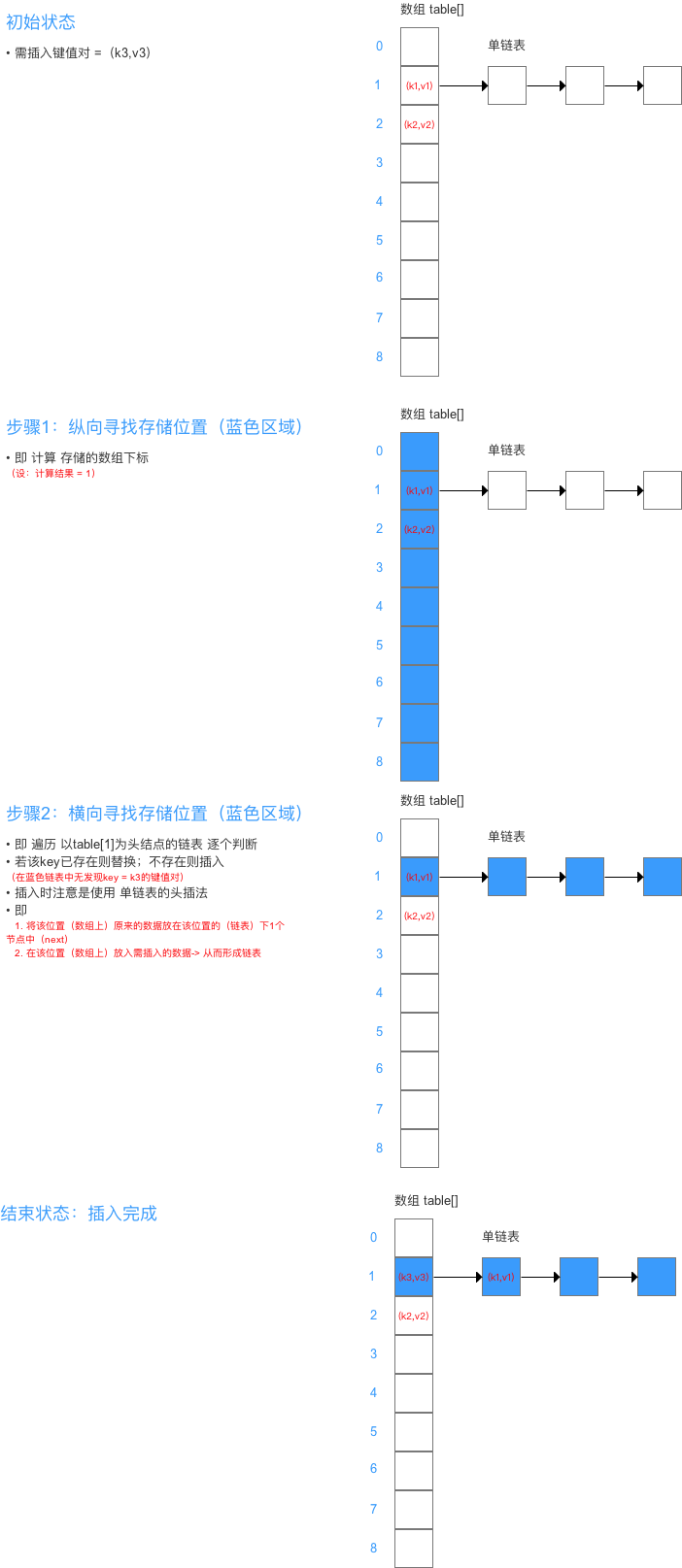### 2. 扩容机制

• 具体流程如下：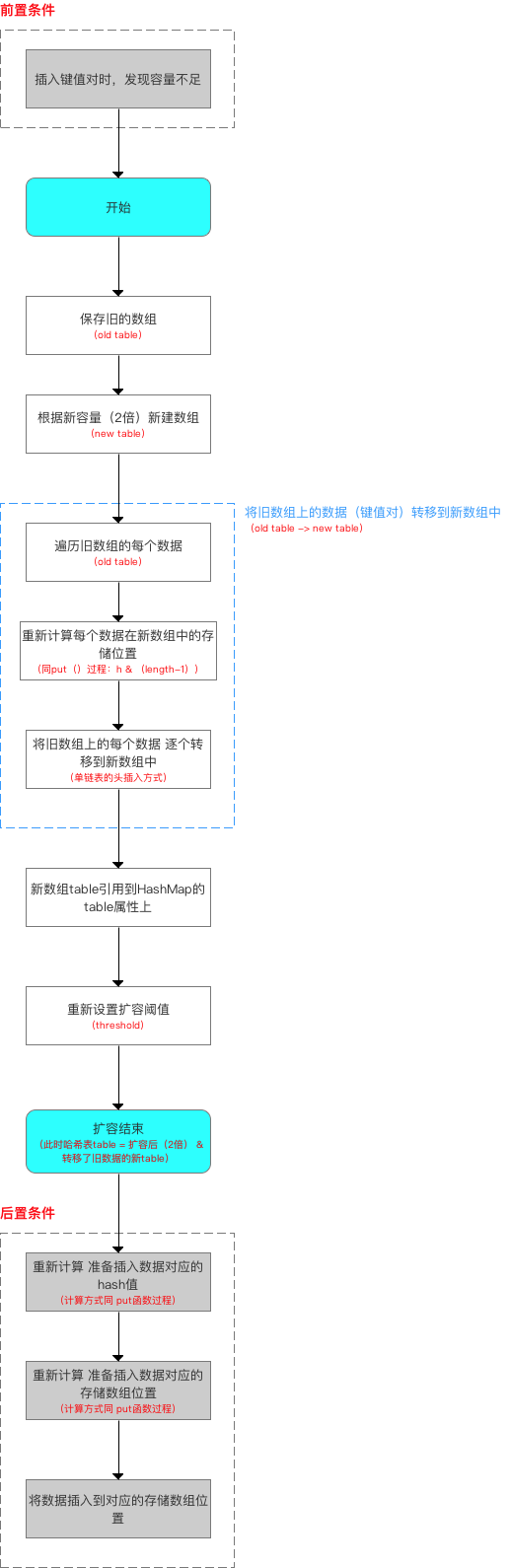• 扩容过程中的转移数据示意图如下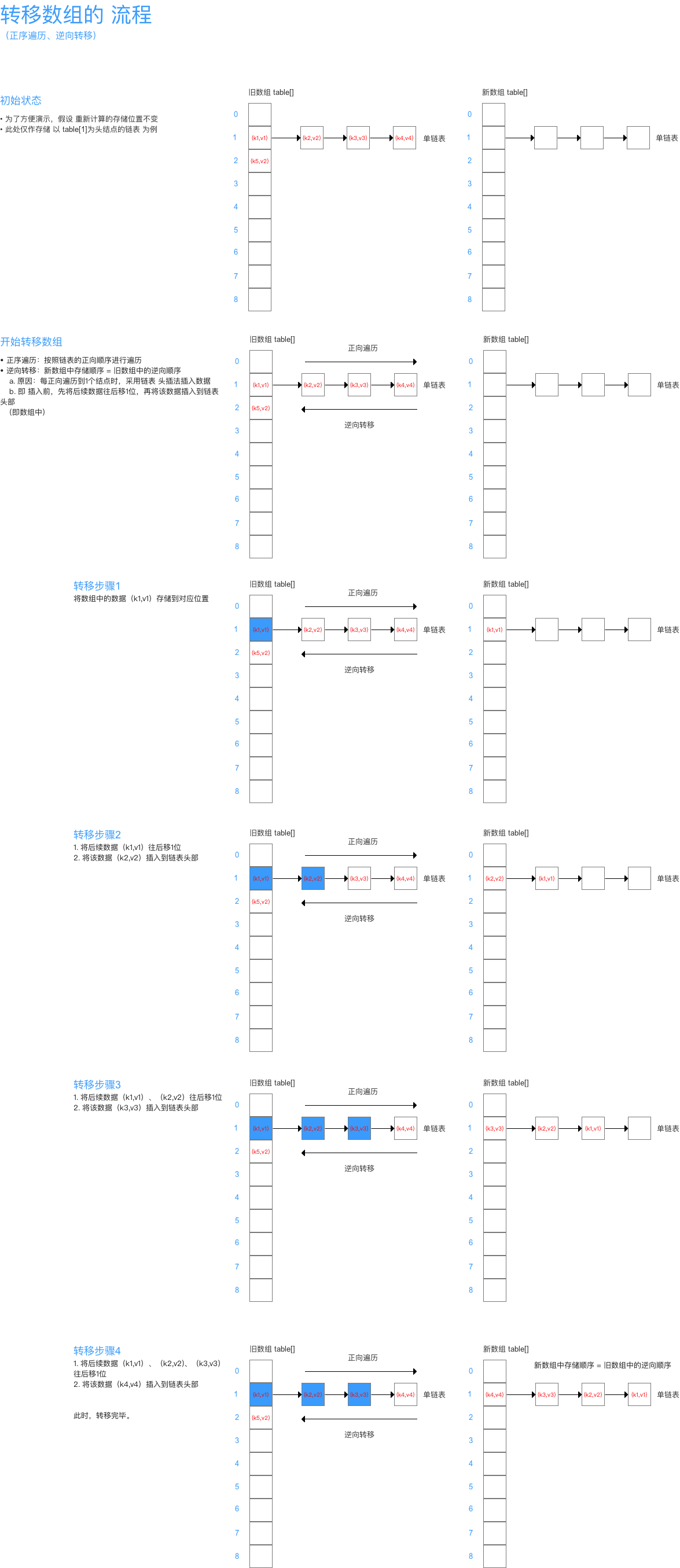• 此时若（多线程）并发执行 put（）操作，一旦出现扩容情况，则 容易出现 环形链表，从而在获取数据、遍历链表时 形成死循环（Infinite Loop），即 死锁的状态 = 线程不安全

### 总结

• HashMap 添加数据（成对 放入 键 - 值对）的全流程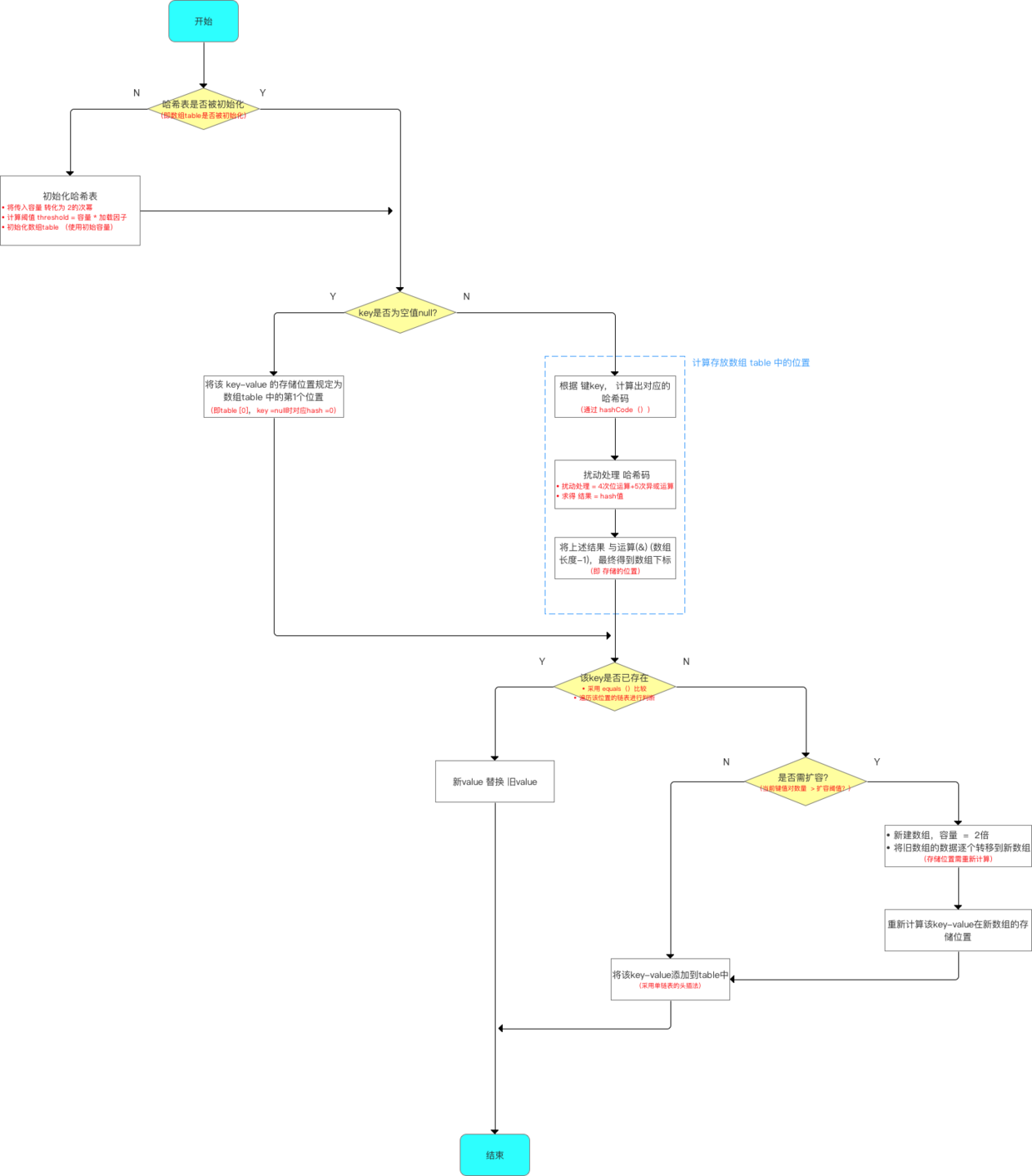• 示意图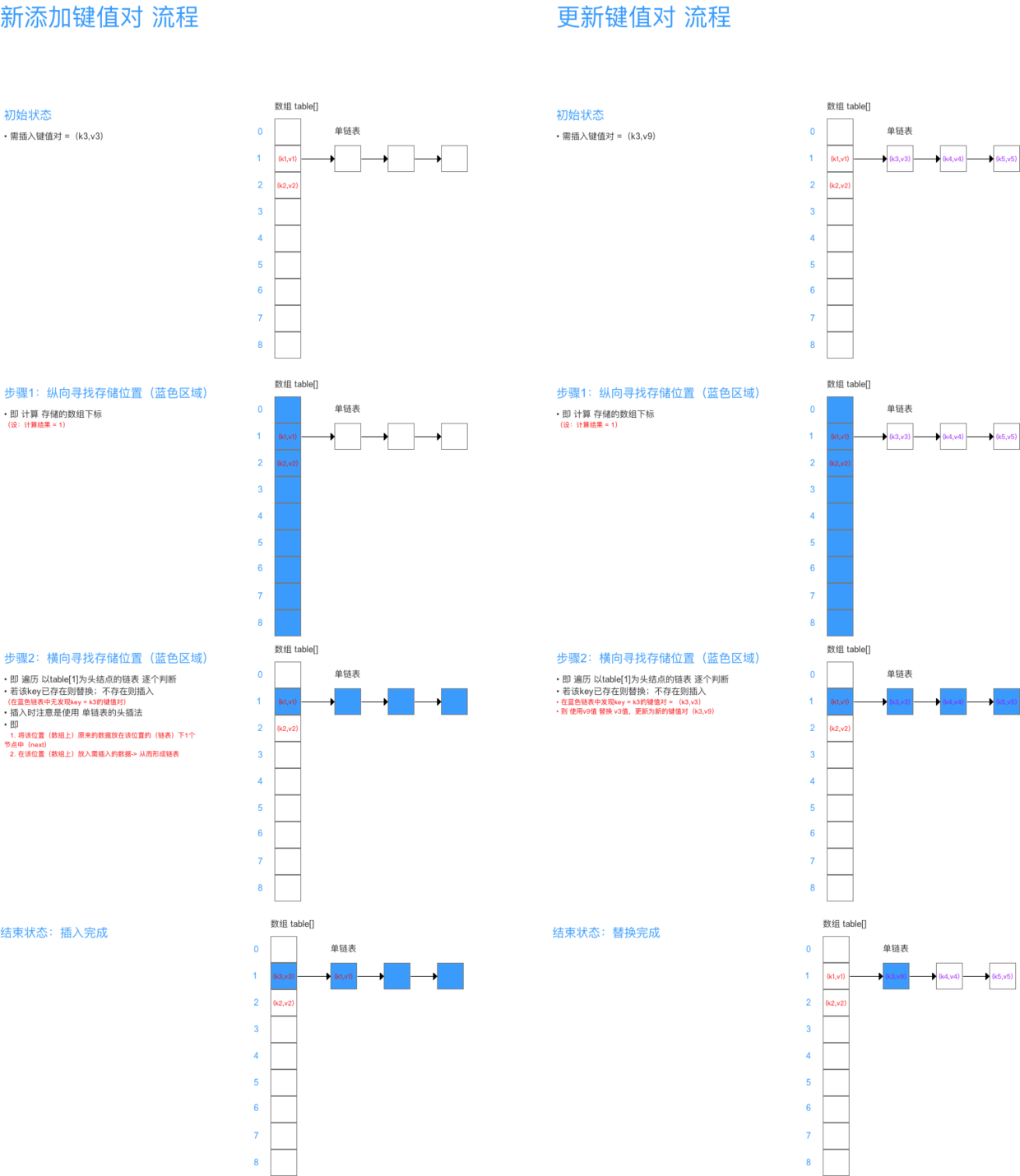# 步骤3：从HashMap中获取数据

• 假如理解了上述put（）函数的原理，那么get（）函数非常好理解，因为二者的过程原理几乎相同
• get（）函数的流程如下：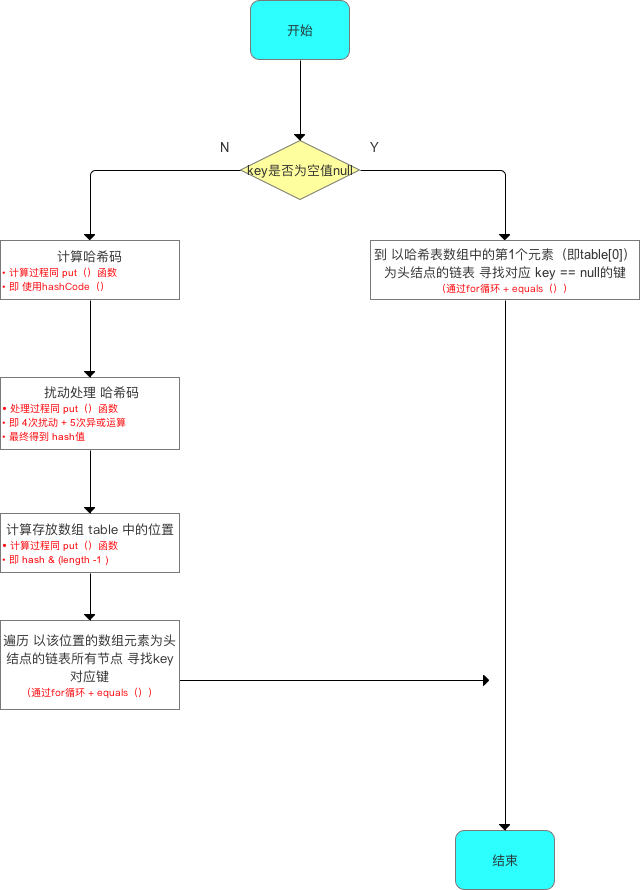• 具体源码分析如下
/**
* 函数原型
* 作用：根据键key，向HashMap获取对应的值
*/
map.get(key)；

/**
* 源码分析
*/
public V get(Object key) {

// 1. 当key == null时，则到 以哈希表数组中的第1个元素（即table）为头结点的链表去寻找对应 key == null的键
if (key == null)
return getForNullKey(); --> 分析1

// 2. 当key ≠ null时，去获得对应值 -->分析2
Entry<K,V> entry = getEntry(key);

return null == entry ? null : entry.getValue();
}

/**
* 分析1：getForNullKey()
* 作用：当key == null时，则到 以哈希表数组中的第1个元素（即table）为头结点的链表去寻找对应 key == null的键
*/
private V getForNullKey() {

if (size == 0) {
return null;
}

// 遍历以table为头结点的链表，寻找 key==null 对应的值
for (Entry<K,V> e = table; e != null; e = e.next) {

// 从table中取key==null的value值
if (e.key == null)
return e.value;
}
return null;
}

/**
* 分析2：getEntry(key)
* 作用：当key ≠ null时，去获得对应值
*/
final Entry<K,V> getEntry(Object key) {

if (size == 0) {
return null;
}

// 1. 根据key值，通过hash（）计算出对应的hash值
int hash = (key == null) ? 0 : hash(key);

// 2. 根据hash值计算出对应的数组下标
// 3. 遍历 以该数组下标的数组元素为头结点的链表所有节点，寻找该key对应的值
for (Entry<K,V> e = table[indexFor(hash, table.length)];  e != null;  e = e.next) {

Object k;
// 若 hash值 & key 相等，则证明该Entry = 我们要的键值对
// 通过equals（）判断key是否相等
if (e.hash == hash &&
((k = e.key) == key || (key != null && key.equals(k))))
return e;
}
return null;
}


### 步骤4：对HashMap的其他操作

• HashMap除了核心的put（）get（）函数，还有以下主要使用的函数方法
void clear(); // 清除哈希表中的所有键值对
int size();  // 返回哈希表中所有 键值对的数量 = 数组中的键值对 + 链表中的键值对
boolean isEmpty(); // 判断HashMap是否为空；size == 0时 表示为 空

void putAll(Map<? extends K, ? extends V> m);  // 将指定Map中的键值对 复制到 此Map中
V remove(Object key);  // 删除该键值对

boolean containsKey(Object key); // 判断是否存在该键的键值对；是 则返回true
boolean containsValue(Object value);  // 判断是否存在该值的键值对；是 则返回true


• 下面将简单介绍上面几个函数的源码分析
  /**
* 函数：isEmpty()
* 作用：判断HashMap是否为空，即无键值对；size == 0时 表示为 空
*/

public boolean isEmpty() {
return size == 0;
}

/**
* 函数：size()
* 作用：返回哈希表中所有 键值对的数量 = 数组中的键值对 + 链表中的键值对
*/

public int size() {
return size;
}

/**
* 函数：clear()
* 作用：清空哈希表，即删除所有键值对
* 原理：将数组table中存储的Entry全部置为null、size置为0
*/
public void clear() {
modCount++;
Arrays.fill(table, null);
size = 0;
}

/**
* 函数：putAll(Map<? extends K, ? extends V> m)
* 作用：将指定Map中的键值对 复制到 此Map中
* 原理：类似Put函数
*/

public void putAll(Map<? extends K, ? extends V> m) {
// 1. 统计需复制多少个键值对
return;

// 2. 若table还没初始化，先用刚刚统计的复制数去初始化table
if (table == EMPTY_TABLE) {
}

// 3. 若需复制的数目 > 阈值，则需先扩容
if (targetCapacity > MAXIMUM_CAPACITY)
targetCapacity = MAXIMUM_CAPACITY;
int newCapacity = table.length;
while (newCapacity < targetCapacity)
newCapacity <<= 1;
if (newCapacity > table.length)
resize(newCapacity);
}
// 4. 开始复制（实际上不断调用Put函数插入）
for (Map.Entry<? extends K, ? extends V> e : m.entrySet())
put(e.getKey(), e.getValue());
}

/**
* 函数：remove(Object key)
* 作用：删除该键值对
*/

public V remove(Object key) {
Entry<K,V> e = removeEntryForKey(key);
return (e == null ? null : e.value);
}

final Entry<K,V> removeEntryForKey(Object key) {
if (size == 0) {
return null;
}
// 1. 计算hash值
int hash = (key == null) ? 0 : hash(key);
// 2. 计算存储的数组下标位置
int i = indexFor(hash, table.length);
Entry<K,V> prev = table[i];
Entry<K,V> e = prev;

while (e != null) {
Entry<K,V> next = e.next;
Object k;
if (e.hash == hash &&
((k = e.key) == key || (key != null && key.equals(k)))) {
modCount++;
size--;
// 若删除的是table数组中的元素（即链表的头结点）
// 则删除操作 = 将头结点的next引用存入table[i]中
if (prev == e)
table[i] = next;

//否则 将以table[i]为头结点的链表中，当前Entry的前1个Entry中的next 设置为 当前Entry的next（即删除当前Entry = 直接跳过当前Entry）
else
prev.next = next;
e.recordRemoval(this);
return e;
}
prev = e;
e = next;
}

return e;
}

/**
* 函数：containsKey(Object key)
* 作用：判断是否存在该键的键值对；是 则返回true
* 原理：调用get（），判断是否为Null
*/
public boolean containsKey(Object key) {
return getEntry(key) != null;
}

/**
* 函数：containsValue(Object value)
* 作用：判断是否存在该值的键值对；是 则返回true
*/
public boolean containsValue(Object value) {
// 若value为空，则调用containsNullValue()
if (value == null)
return containsNullValue();

// 若value不为空，则遍历链表中的每个Entry，通过equals（）比较values 判断是否存在
Entry[] tab = table;
for (int i = 0; i < tab.length ; i++)
for (Entry e = tab[i] ; e != null ; e = e.next)
if (value.equals(e.value))
return true;//返回true
return false;
}

// value为空时调用的方法
private boolean containsNullValue() {
Entry[] tab = table;
for (int i = 0; i < tab.length ; i++)
for (Entry e = tab[i] ; e != null ; e = e.next)
if (e.value == null)
return true;
return false;
}



# 6. 源码总结

• 数据结构 & 主要参数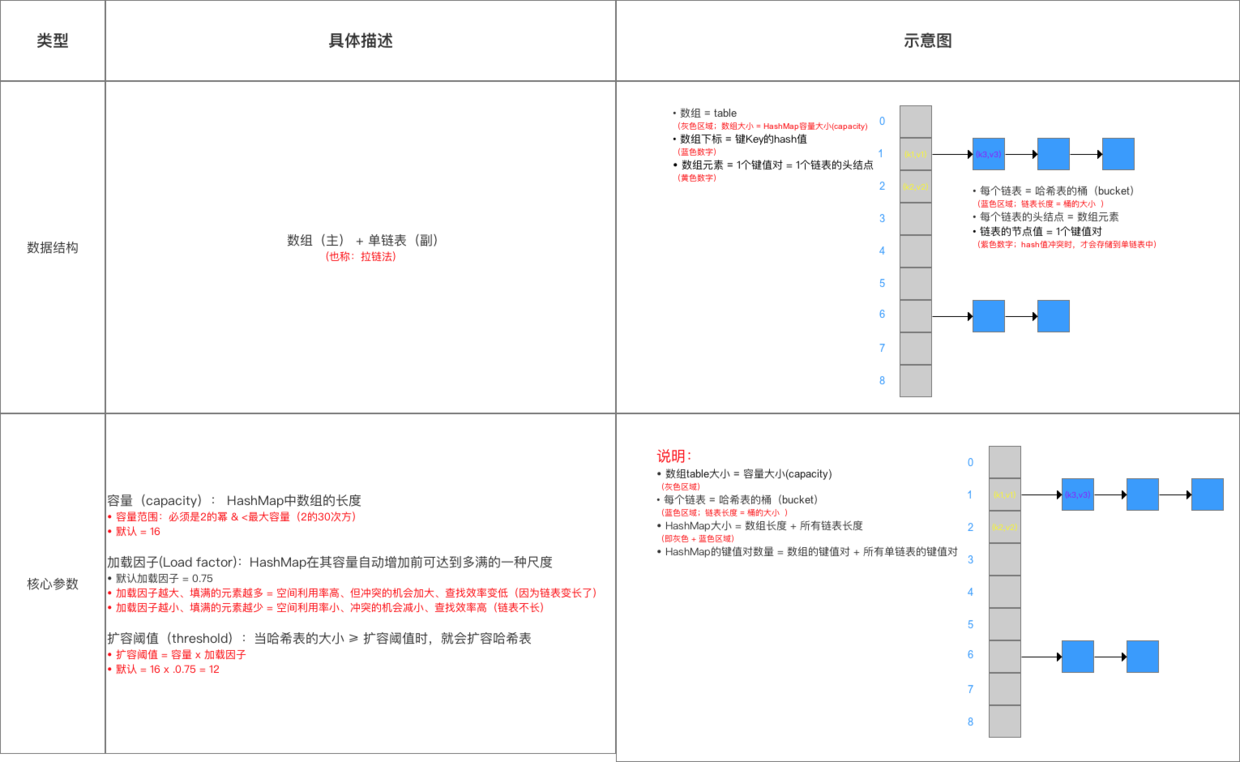• 添加 & 查询数据流程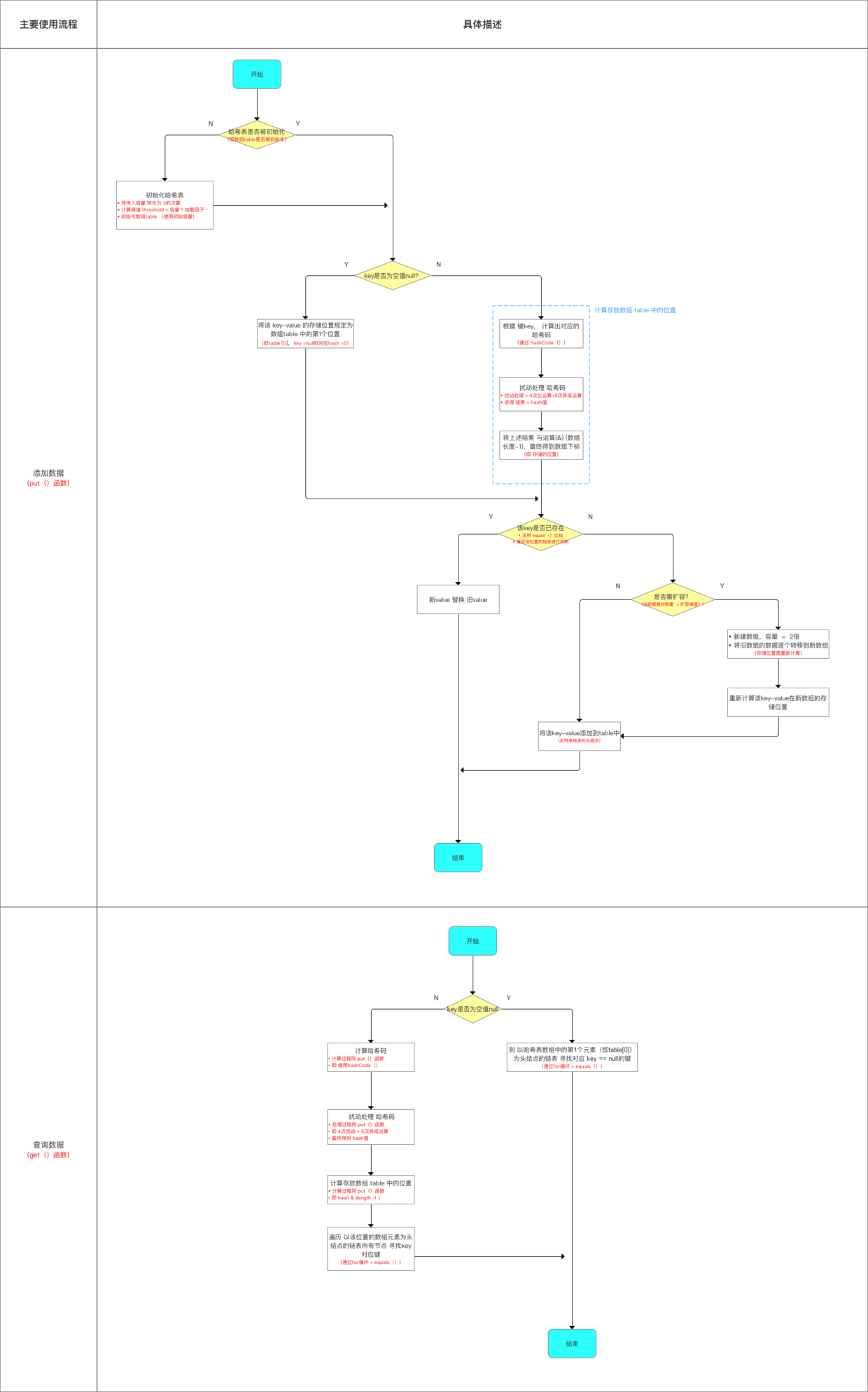• 扩容机制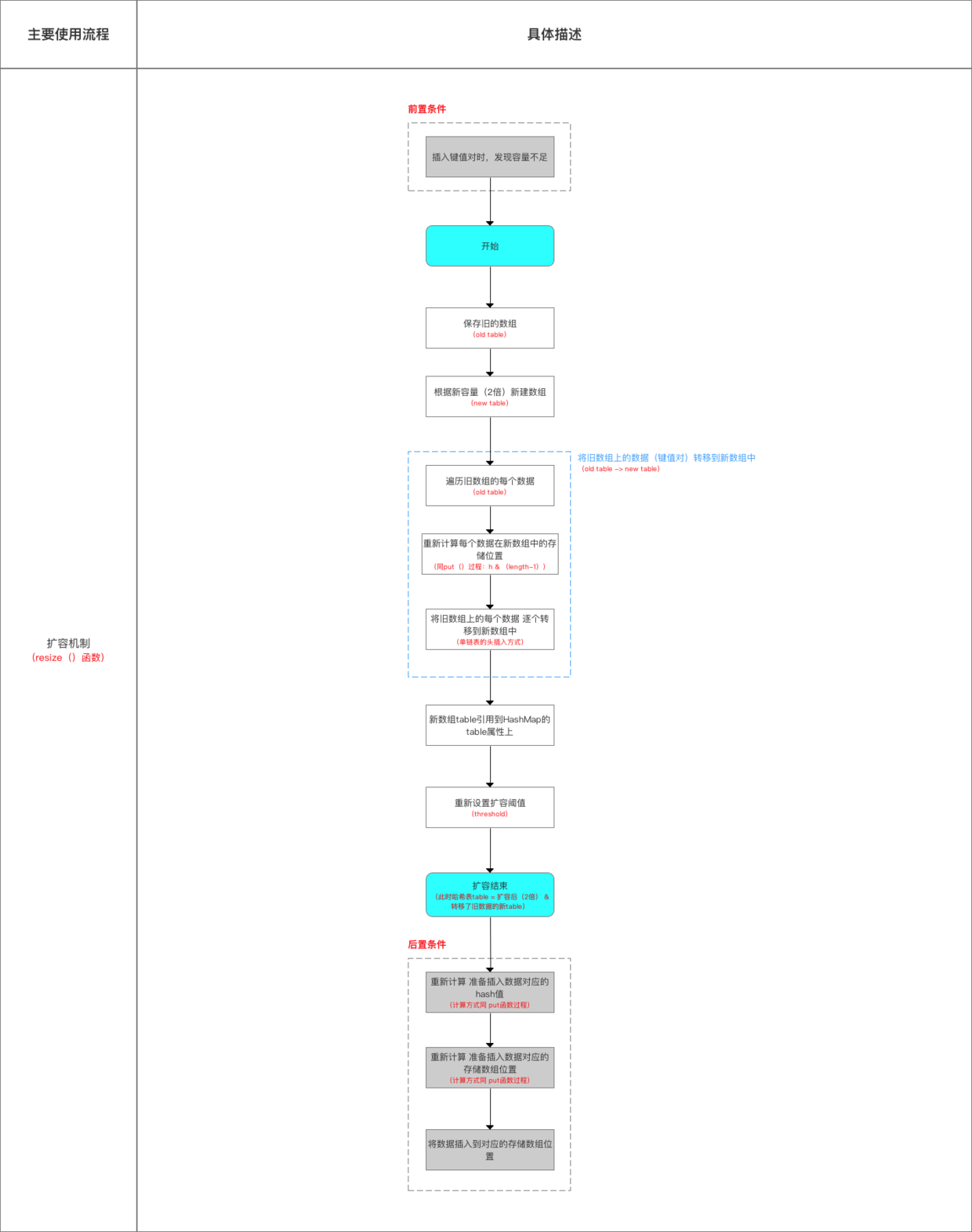# 7. 与 JDK 1.8的区别

HashMap 的实现在 JDK 1.7JDK 1.8 差别较大，具体区别如下

JDK 1.8 的优化目的主要是：减少 Hash冲突 & 提高哈希表的存、取效率；关于 JDK 1.8HashMap 的源码解析请看文章：Java源码分析：关于 HashMap 1.8 的重大更新

### 7.1 数据结构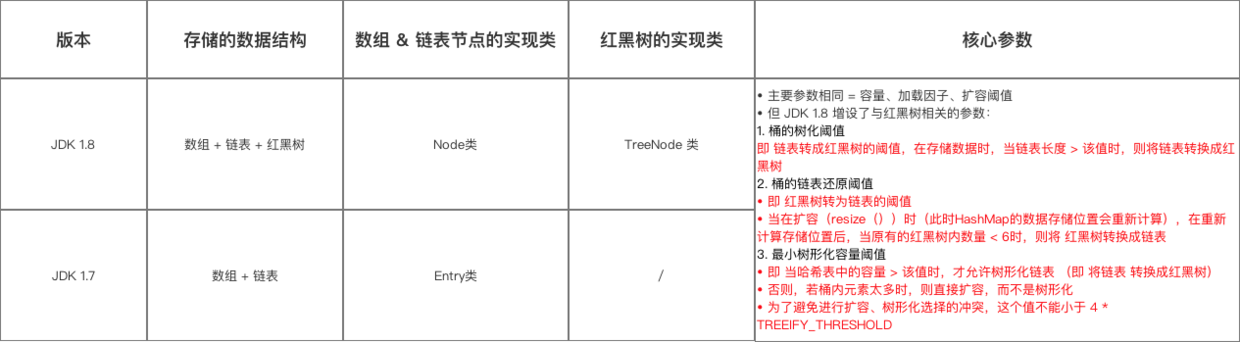### 7.2 获取数据时（获取数据 类似）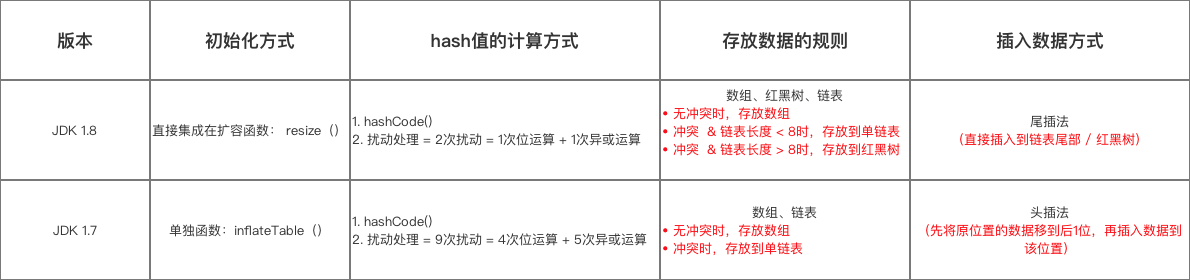### 7.3 扩容机制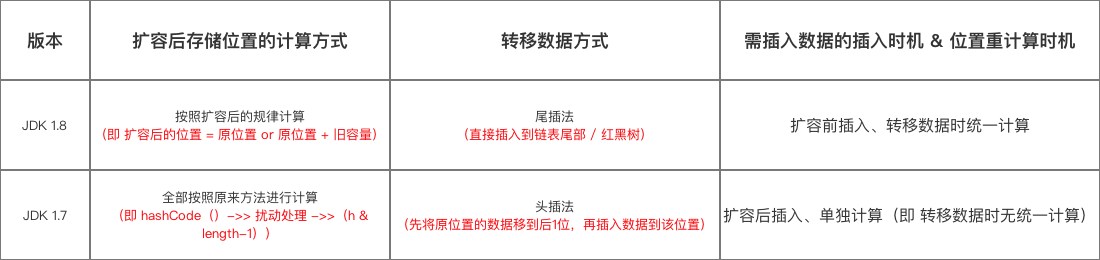# 8. 额外补充：关于HashMap的其他问题

• 有几个小问题需要在此补充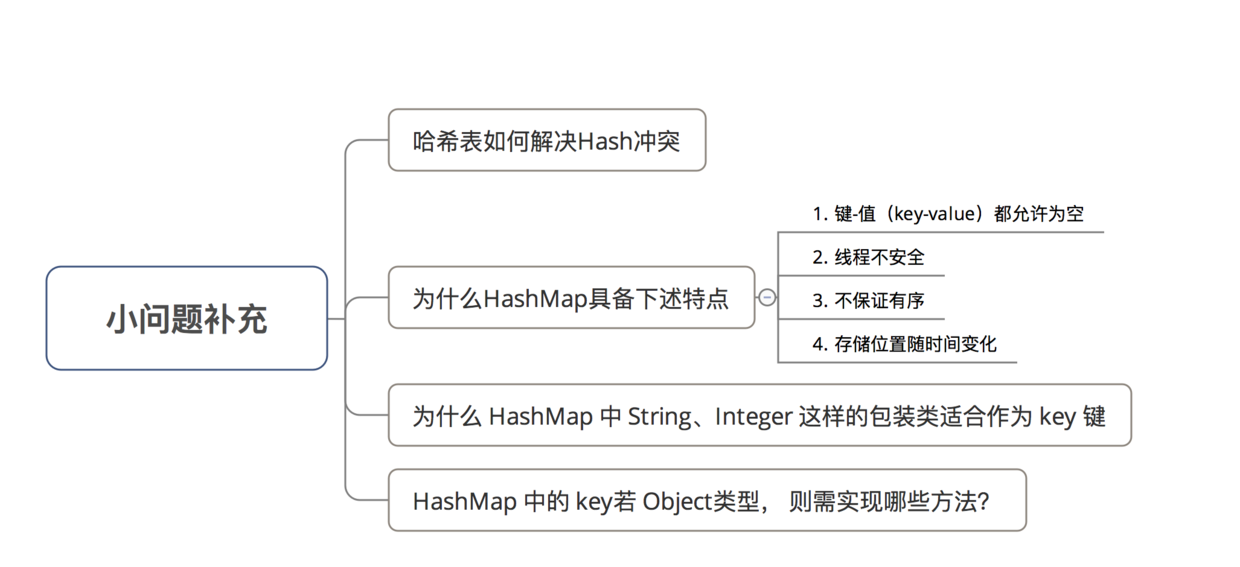• 具体如下

### 8.1 哈希表如何解决Hash冲突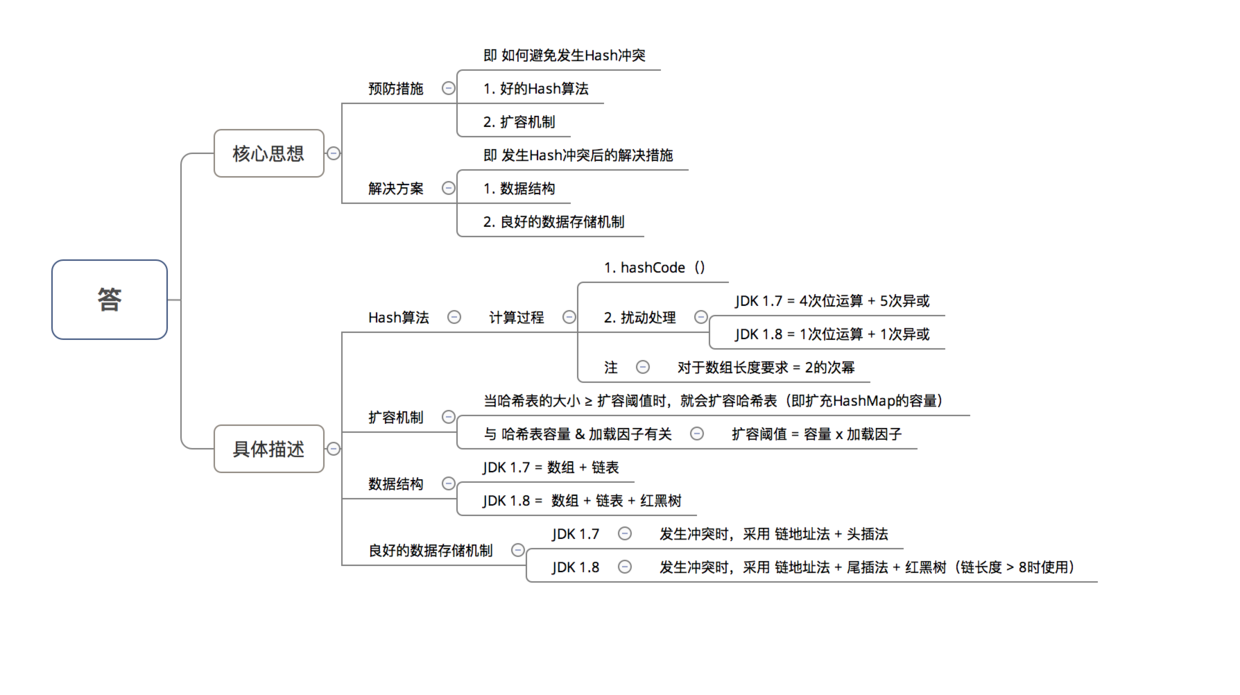### 8.2 为什么HashMap具备下述特点：键-值（key-value）都允许为空、线程不安全、不保证有序、存储位置随时间变化

• 具体解答如下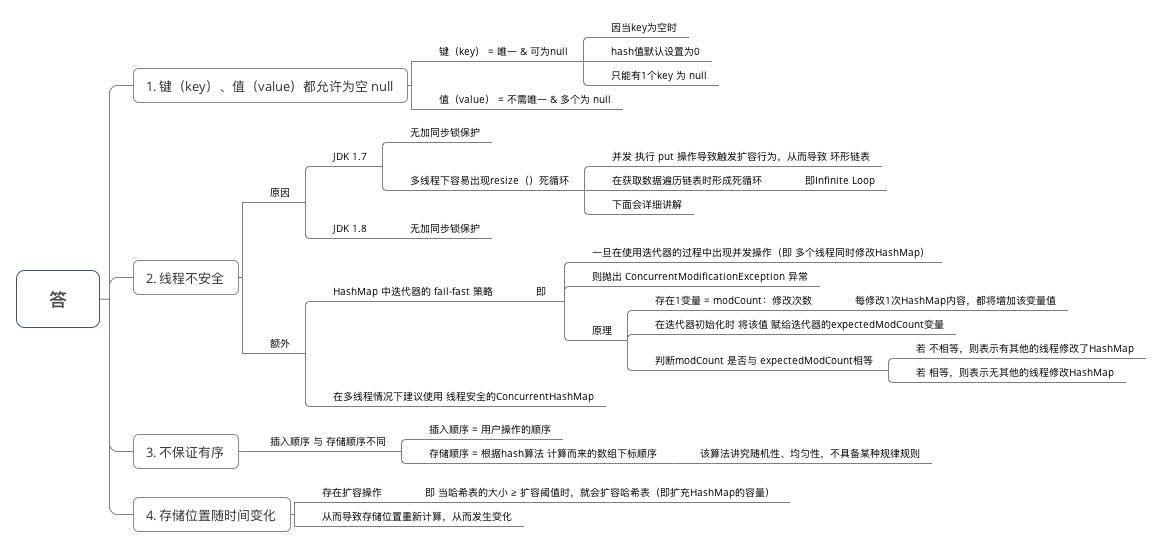• 下面主要讲解 HashMap 线程不安全的其中一个重要原因：多线程下容易出现resize（）死循环
本质 = 并发 执行 put（）操作导致触发 扩容行为，从而导致 环形链表，使得在获取数据遍历链表时形成死循环，即Infinite Loop

• 先看扩容的源码分析resize（）

/**
* 源码分析：resize(2 * table.length)
* 作用：当容量不足时（容量 > 阈值），则扩容（扩到2倍）
*/
void resize(int newCapacity) {

// 1. 保存旧数组（old table）
Entry[] oldTable = table;

// 2. 保存旧容量（old capacity ），即数组长度
int oldCapacity = oldTable.length;

// 3. 若旧容量已经是系统默认最大容量了，那么将阈值设置成整型的最大值，退出
if (oldCapacity == MAXIMUM_CAPACITY) {
threshold = Integer.MAX_VALUE;
return;
}

// 4. 根据新容量（2倍容量）新建1个数组，即新table
Entry[] newTable = new Entry[newCapacity];

// 5. （重点分析）将旧数组上的数据（键值对）转移到新table中，从而完成扩容 ->>分析1.1
transfer(newTable);

// 6. 新数组table引用到HashMap的table属性上
table = newTable;

// 7. 重新设置阈值
}

/**
* 分析1.1：transfer(newTable);
* 作用：将旧数组上的数据（键值对）转移到新table中，从而完成扩容
* 过程：按旧链表的正序遍历链表、在新链表的头部依次插入
*/
void transfer(Entry[] newTable) {
// 1. src引用了旧数组
Entry[] src = table;

// 2. 获取新数组的大小 = 获取新容量大小
int newCapacity = newTable.length;

// 3. 通过遍历 旧数组，将旧数组上的数据（键值对）转移到新数组中
for (int j = 0; j < src.length; j++) {
// 3.1 取得旧数组的每个元素
Entry<K,V> e = src[j];
if (e != null) {
// 3.2 释放旧数组的对象引用（for循环后，旧数组不再引用任何对象）
src[j] = null;

do {
// 3.3 遍历 以该数组元素为首 的链表
// 注：转移链表时，因是单链表，故要保存下1个结点，否则转移后链表会断开
Entry<K,V> next = e.next;
// 3.3 重新计算每个元素的存储位置
int i = indexFor(e.hash, newCapacity);
// 3.4 将元素放在数组上：采用单链表的头插入方式 = 在链表头上存放数据 = 将数组位置的原有数据放在后1个指针、将需放入的数据放到数组位置中
// 即 扩容后，可能出现逆序：按旧链表的正序遍历链表、在新链表的头部依次插入
e.next = newTable[i];
newTable[i] = e;
// 访问下1个Entry链上的元素，如此不断循环，直到遍历完该链表上的所有节点
e = next;
} while (e != null);
// 如此不断循环，直到遍历完数组上的所有数据元素
}
}
}


• 此时若（多线程）并发执行 put（）操作，一旦出现扩容情况，则 容易出现 环形链表，从而在获取数据、遍历链表时 形成死循环（Infinite Loop），即 死锁的状态，具体请看下图：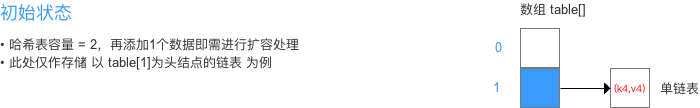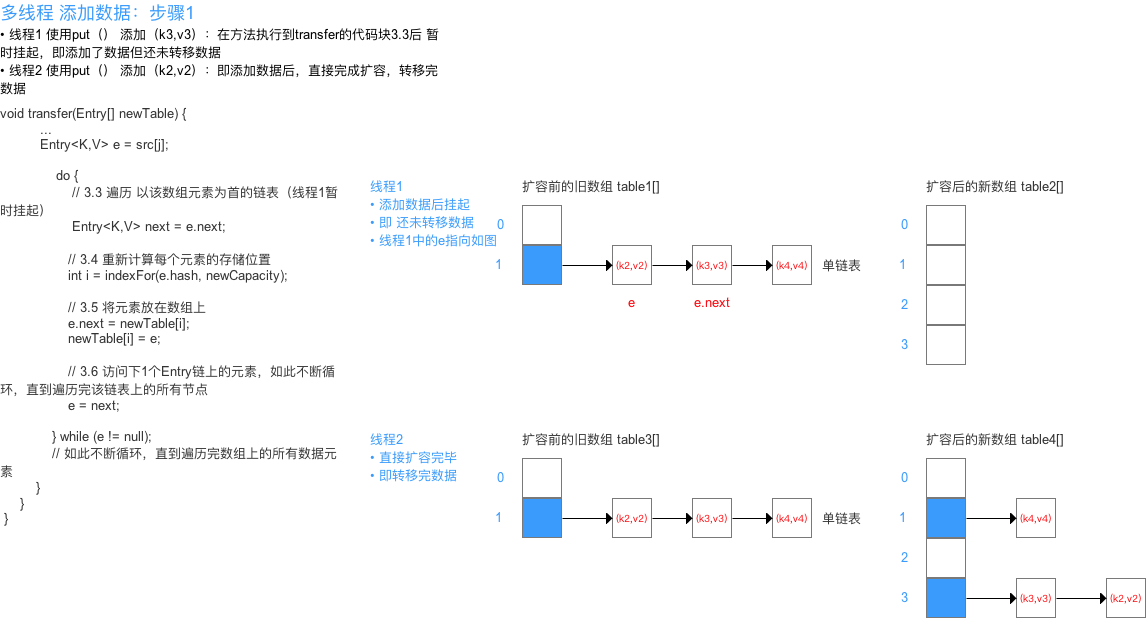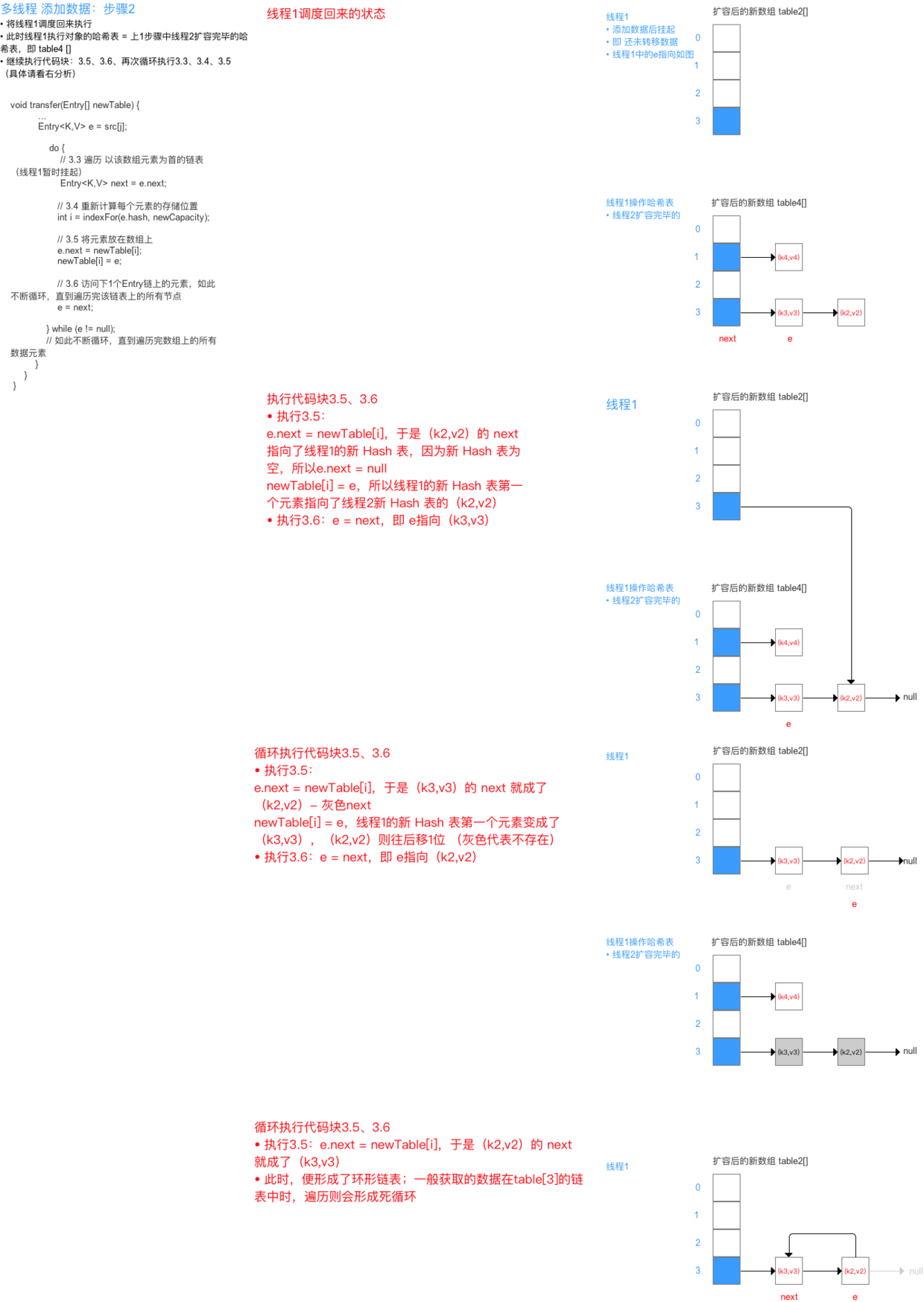JDK 1.8 还是线程不安全，因为 无加同步锁保护

### 8.3 为什么 HashMap 中 String、Integer 这样的包装类适合作为 key 键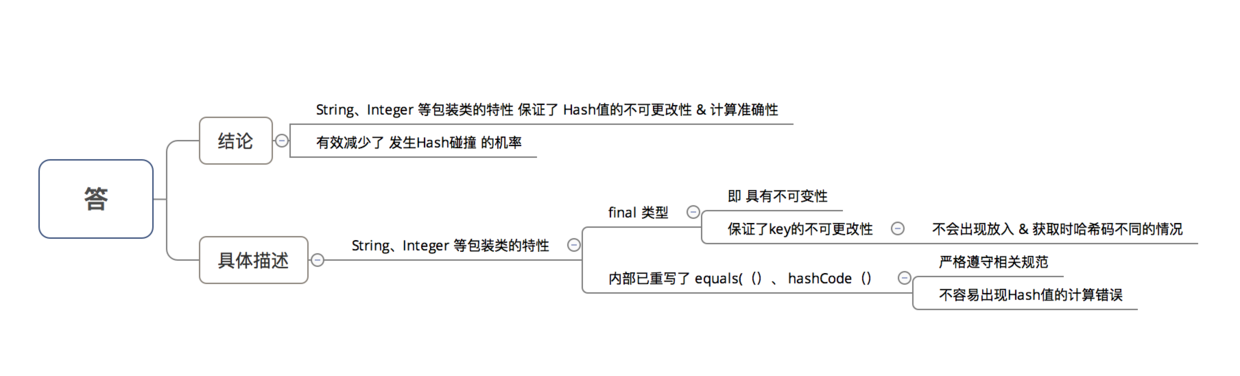### 8.4 HashMap 中的 key若 Object类型， 则需实现哪些方法？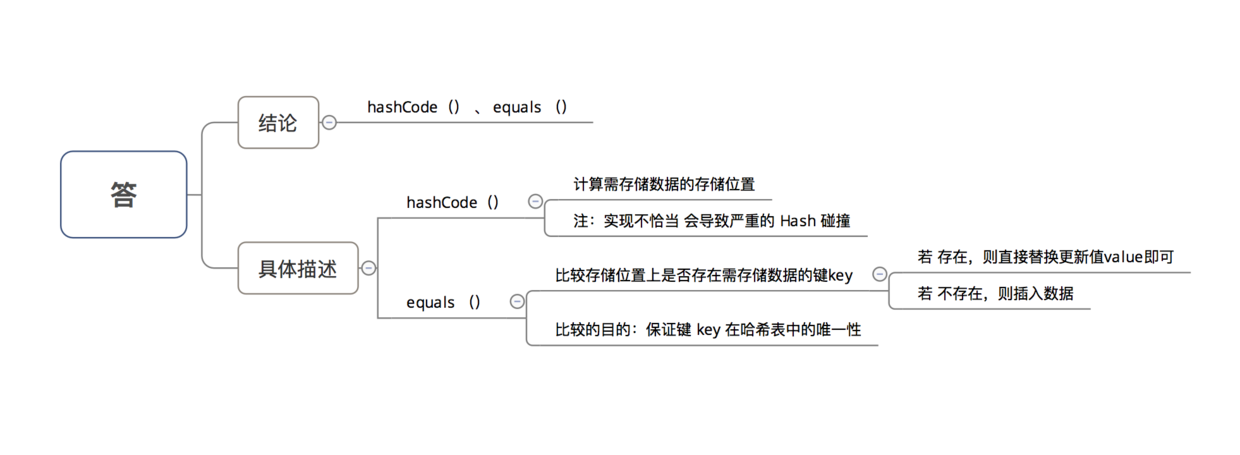# 9. 总结

• 本文主要讲解 JavaHashMap源码 & 相关知识
• 下面我将继续对JavaAndroid中的其他知识 深入讲解 ，感兴趣的同学可以继续关注carson_ho的微信公众号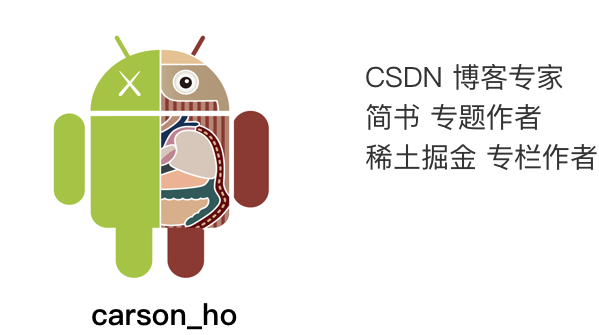# 请帮顶 / 评论点赞！因为你的鼓励是我写作的最大动力！

0 个人打赏©️2019 CSDN 皮肤主题: 编程工作室 设计师: CSDN官方博客